• 考研平时考试必备的公式总结 考研平时考试必备的公式总结
• 信号与系统：①信号与系统的基本知识；②连续信号与系统的时域分析；③信号与系统的变换域分析；④离散信号与系统时域分析；⑤系统函数；⑥信号与系统的状态变量分析，包含书本中所需公式，便于查看，学习
• 信号与系统公式总结，文件分为两部分，前半部分49页，利用表格对信号系统的所有常见不常见公式名字、符号、性质、响应特性、连续离散、易错易混知识点对比等做了展现。后半部分41页，对卷积、响应激励、傅里叶变换、...
• 信号与系统是各高等院校电子类专业和通信类专业学生的一门非常重要的专业基础课。信号与系统概念公式总结.
• 信号与系统公式方法和总结~~很不错的，需要的去下载哈
• 包含信号与系统自己总结部分公式！不太会用编辑器 后面继续上传
• 吴大正版的每章的公式总结并附有两套试卷 比较系统，清晰的
• 自己总结的一些信号与线性系统的常用公式，包括傅里叶变换，S域变换，Z域变换，离散傅里叶变换等，卷积公式，供大家参考学习。
• 信号与系统》常用公式整理，包括傅里叶变换、拉普拉斯变换、z变换。三大变换统一整理，非常适合考研党。
• ## 信号与系统实用总结

万次阅读 多人点赞 2017-02-13 23:04:39
信号与系统是个比较抽象的课，因为它是用数学建模的方法去分析电路。
那么，我们把电路的现象结合信号与系统的理论指导，就会发现柳暗花明又一村。
1、什么是信号？什么是噪声？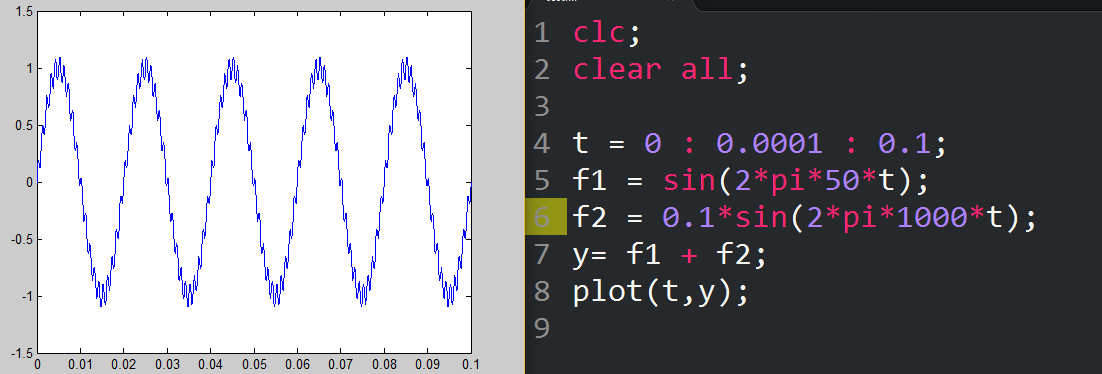没错，出来的波形并不是我们想要的光滑的50Hz正弦波，而是有一点奇葩的波形。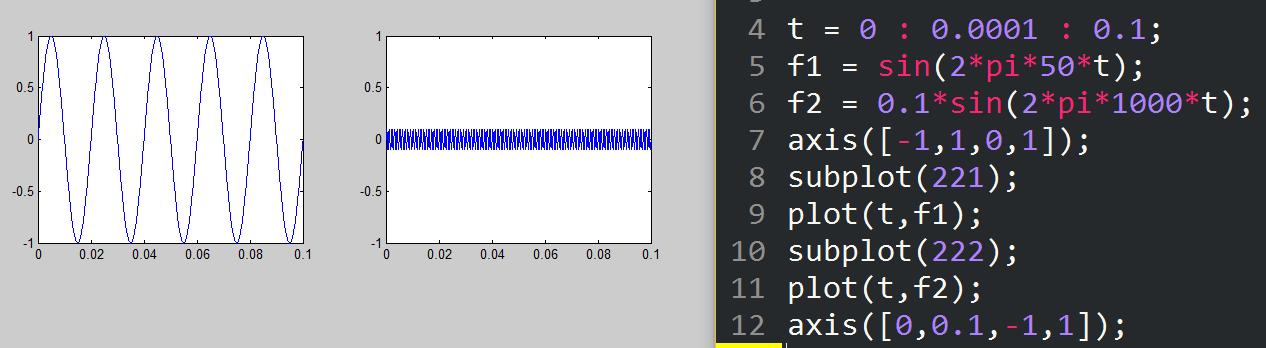经过分析得到，奇葩的波形，是由50Hz正弦波和1KHz的正弦波叠加而成，但是1KHz的正弦波并不是我们想要的东西。
综上所述，你想要的东西就是信号（50Hz），不想要的东西（1KHz）就是噪声。当然，前提是你要清楚你想要的东西是什么。
BTW，信号不仅仅包含电信号，也包含声音、光、热、力等等。
2、什么是系统？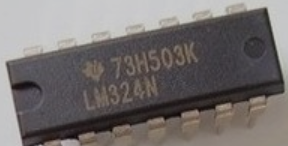LM324这种运放，就是一个系统。我们不能知道它的内部构造，但是我们可以搭个电路，从外部去测量它的性能。
这就是信号与系统，最重要的思想。把系统当成一个黑匣子，测量外部的信号，从而评估系统的特性。
因为，很多时候，我们不需要知道系统的内部构造。
BTW，系统不仅仅包含运放，小到一个电容，大到整个电路板，任何我们想观察的事物，都可以称为系统。
3、什么是激励？什么是响应？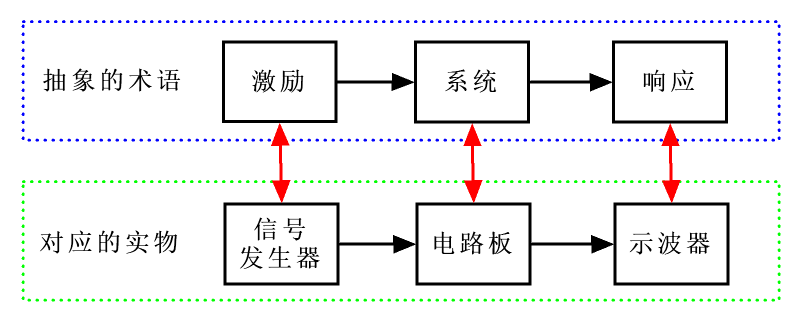信号与系统最基本的研究方法就是，用信号发生器送信号到电路板，再用示波器去观察输出的波形。
这种操作，也就是送激励到系统，再观察响应。
如果利用信号发生器扫频的功能，再配合示波器，很容易就得到电路板的幅频特性和相频特性。这就非常有助我们去理解这个电路板的性能。
很多时候我们不关心系统的响应，只关心系统的稳定性。
也没那么多时间去扫频，而且扫频并不是十分方便。所以人们想到一个方法，利用一些频率成份特别丰富的激励去测试系统的稳定性（其中一个频率成份使得系统自激，就说明不稳定，如：运放等电路）。
最常用的激励有：冲激函数、窗函数和阶跃函数。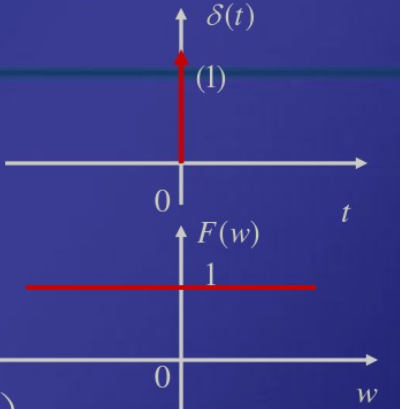上图分别为冲激函数的时域图和频谱。显然，冲激函数的频谱是无限广，也就是包含无数个频率成分。
但是冲激函数在物理上不无法实现的。所以人们想到用一个精度足够高的窄脉冲代替冲激函数。这个窄脉冲就是窗函数了。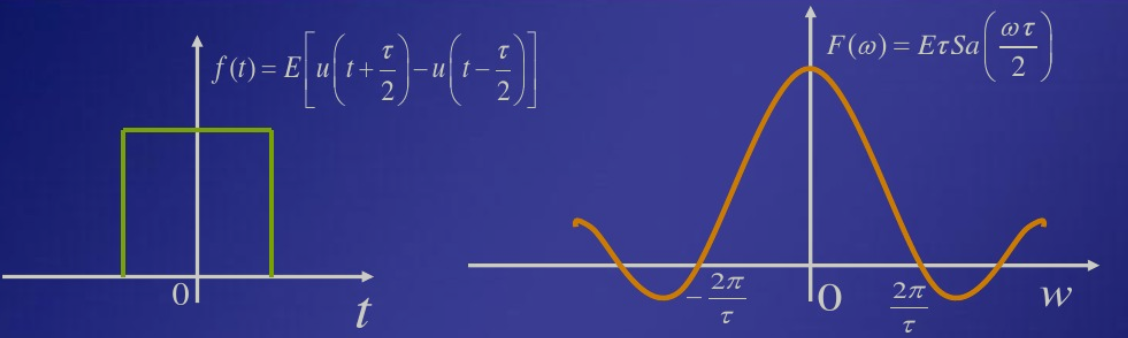上图分别为窗函数的时域图和频谱。显然，窗函数的频谱也是无限广，但是更容易现实。
如果没法弄到窄函数，那么，在要求不是很高的情况下，用一个简单的开关动作去产生阶跃函数也是可以的。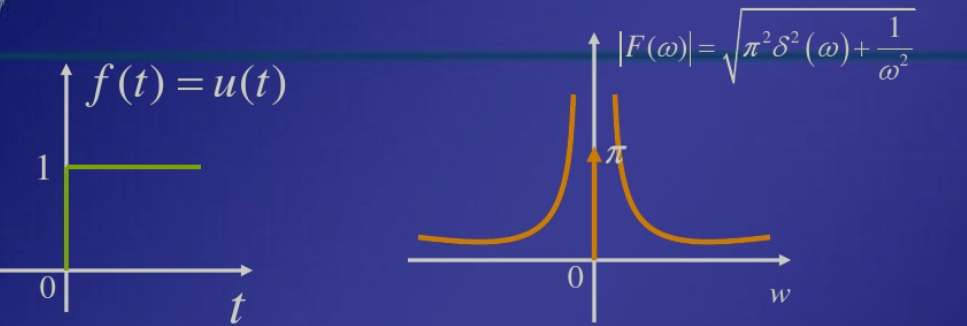上图分别为阶跃函数的时域图和频谱。阶跃函数的频谱也是很广的，虽然比不上冲激函数和窗函数。
4、什么是卷积？
卷积是一种积分，既然是积分，就会有先分割再叠加的操作。如下图所示。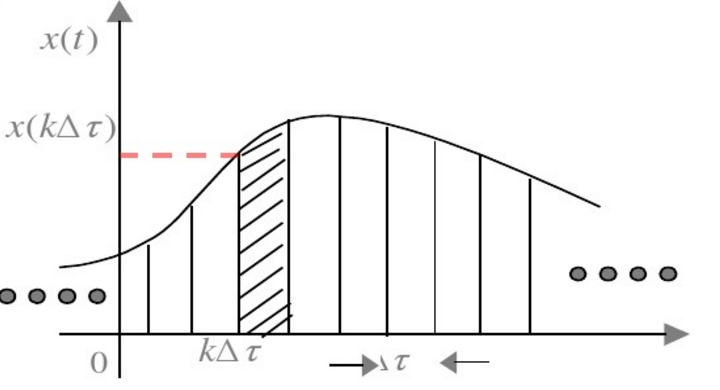那么卷积就是先把输入信号分割成若干个单位冲激信号，再作用于系统函数（即加权），最后再叠加。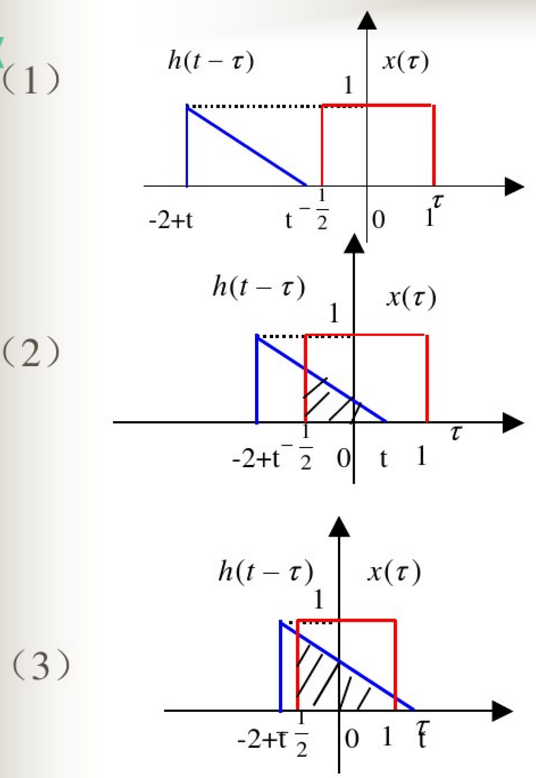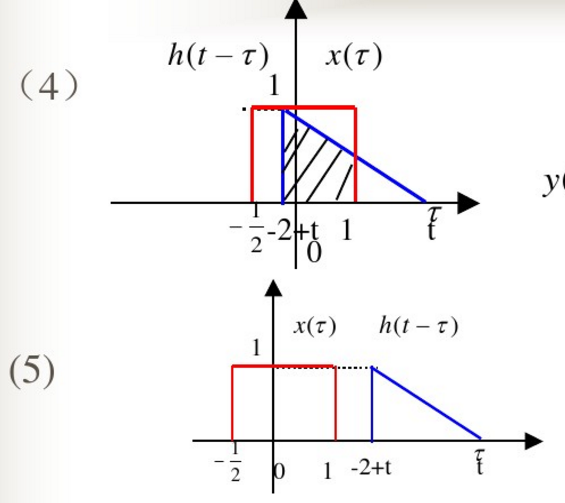把上图中步骤(1)~(5)的阴影部分累加起来，就得到输出信号。如下图所示。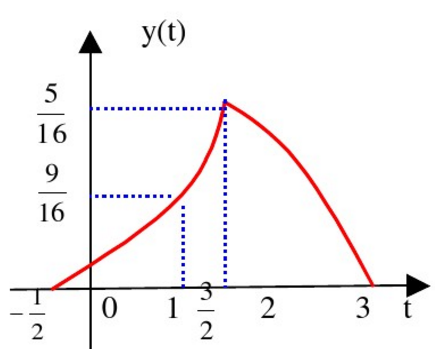于是，我们得到卷积的计算公式。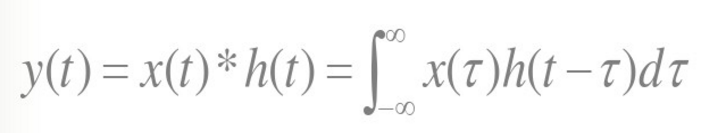一看，发现没有冲激信号啊，其实上面的公式是一个简化后的公式，它把冲激信号隐藏起来了。
先看看冲激信号的筛选性质。如下图所示。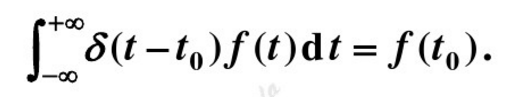把一个信号分解成若干个冲激信号，再叠加，其实还是原来的信号，所以卷积中仍然使用h(t-τ)，
但是t-τ是冲激信号的延时性质遗留下来的，把h(t)延时τ就得到了h(t-τ)。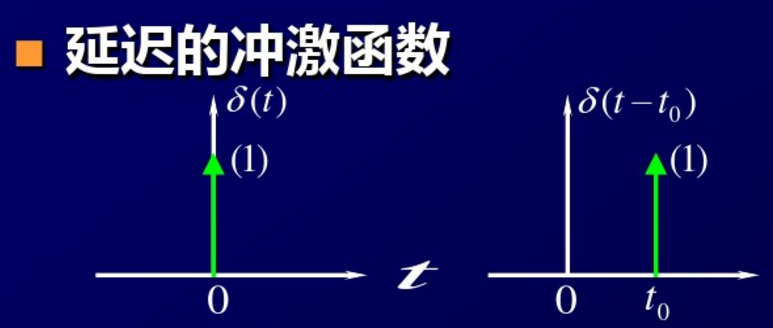为什么要把信号延时、平移？
先延时再平移，才让输入信号和系统函数之间有交集，发生作用。否则的话，哪来输出？
前面延时t为变量τ为常量，现在平移τ为变量t为常量。
τ的取值范围是正负无穷，也就是说把h(t)从负无穷平移到正无穷，所以总能使用输入信号和系统函数产生交集，最终得到输出。
为什么要把信号翻转？
跟时间有关。
我们在图上看到的h(t)函数，把t由小到大排列是h(0)、h(1)、h(2)。。。
但是，细想一下哪个时刻最先出现？没错，是h(0)，所以才要把信号翻转一下，使得h(0)最先起作用。
所以利用图解法解释卷积时，一上来就把信号反转，让人有点奇怪。
卷积定理揭示了时域和频域之间的关系，是一个非常重要的定理，这里提一下。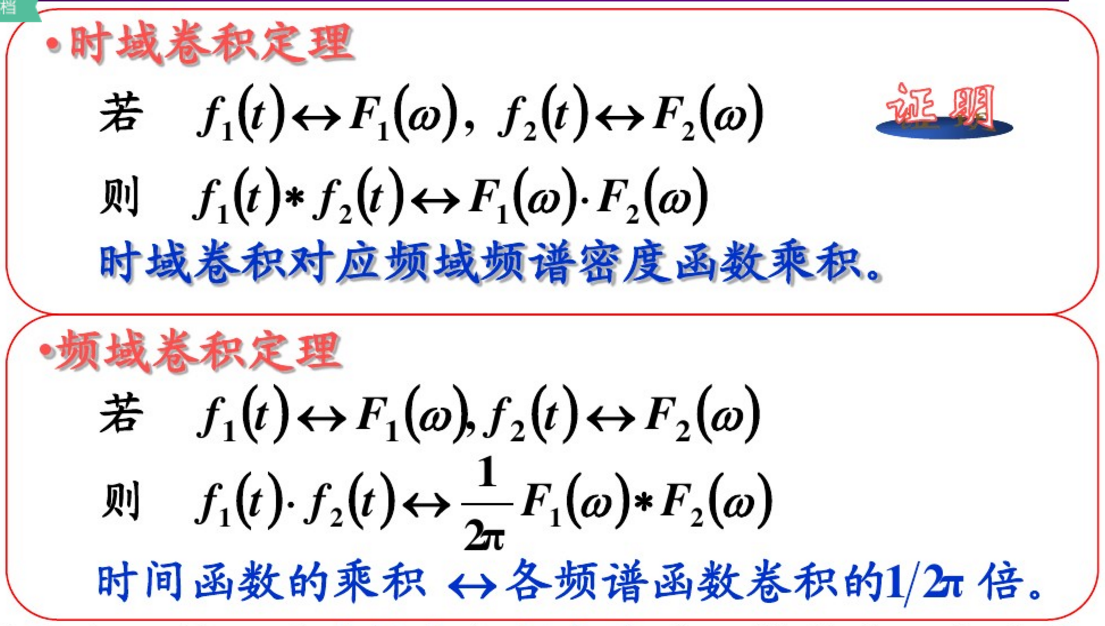5、什么是傅里叶级数？什么是傅里叶变换？
请认真地看《如果看了这篇文章你还不懂傅里叶变换,那就过来掐死我吧》。
这里不再重复。
BTW，一般的数字示波器都有一个FFT的功能，可以看到信号的频谱，这就非常有利于我们分析信号的频率成份。
6、什么是拉普拉斯变换？
拉普拉斯变换又称拉氏变换。在电学里面，拉普拉斯变换只是作为求解微分方程的一种简单方法，属于积分变换法。
拉普拉斯变换可以把复杂的微分方程转化为线性方程。
利用拉普拉斯变换可以求解各种复杂电路的响应，包括各种的运放电路。如下图所示。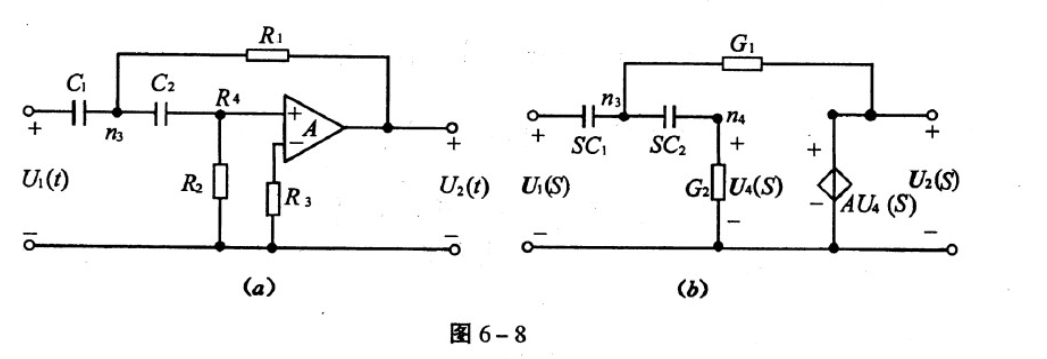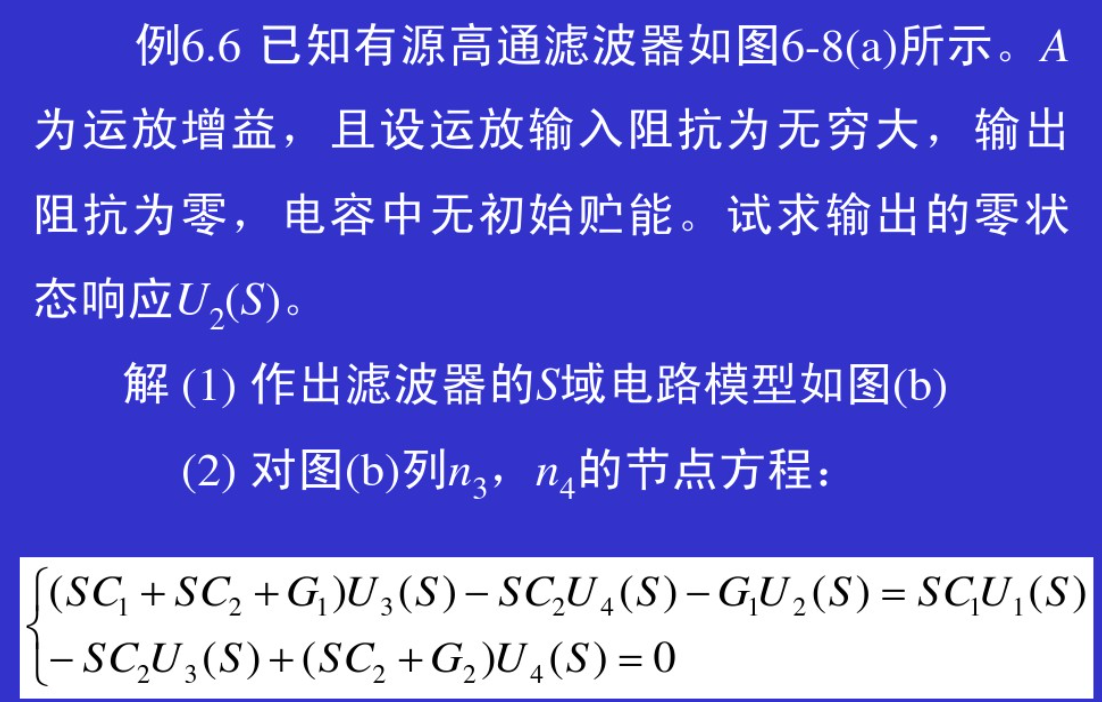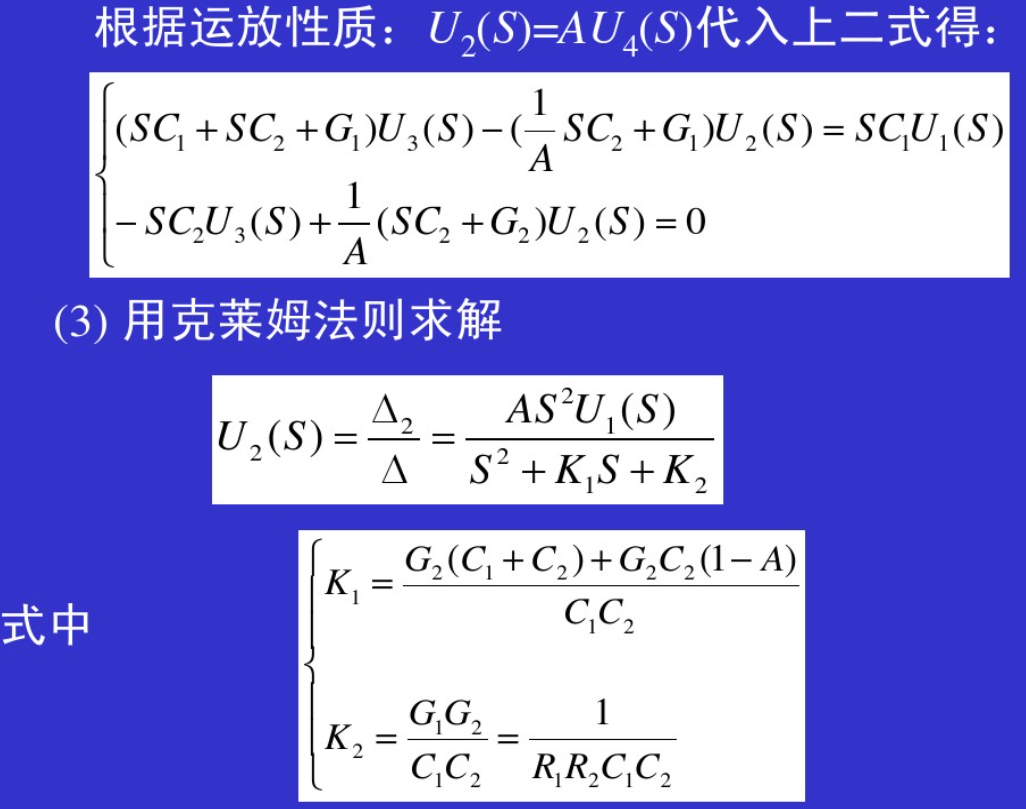很多人认为拉普拉斯变换没用，其实是仿真工具用了。
熟练使用拉普拉斯变换，有利于理解电路的稳定性、响应，可以提高硬件设计能力。
7、什么是Z变换？
拉普拉斯变换适用于连续信号，但是计算机难以处理连续信号（无数个点）。
把连续信号采样后，再做z变换，所有的计算变成有限个点，这样才能送到计算机中处理。z变换也就是离散信号的拉普拉斯变换。
z变换和拉普拉斯在定义、性质上都是大同小异，这里不再重复。
特别需要指出，z的-1次方，其实就是对应于一个D触发器，可以延时一个时钟周期。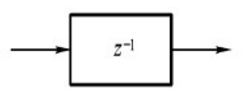而z的2次方，是超前2个时钟周期，不易于用物理实现，那么，你可以把超前换成滞后（整个电路滞后3拍），就能转化为D触发器了。
8、傅里叶变换、拉普拉斯变换、z变换的关系。
(1) 连续信号使用傅里叶变换，必须满足绝对可积的条件。
(2) 离散信号使用傅里叶变换，必须满足绝对可和的条件。
这两个条件都是比较苛刻的，对于一些不满足条件的信号将无法使用傅里叶变换来分析。
所以，需要将傅里叶变换扩展到s平面，即拉普拉斯变换，这样可以分析那些不满足条件的信号。
而z变换是用于分析离散信号，s平面与z平面的关系：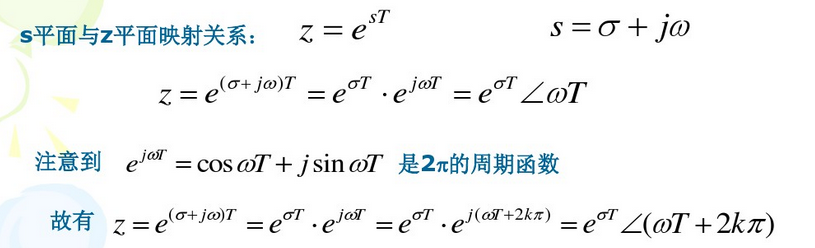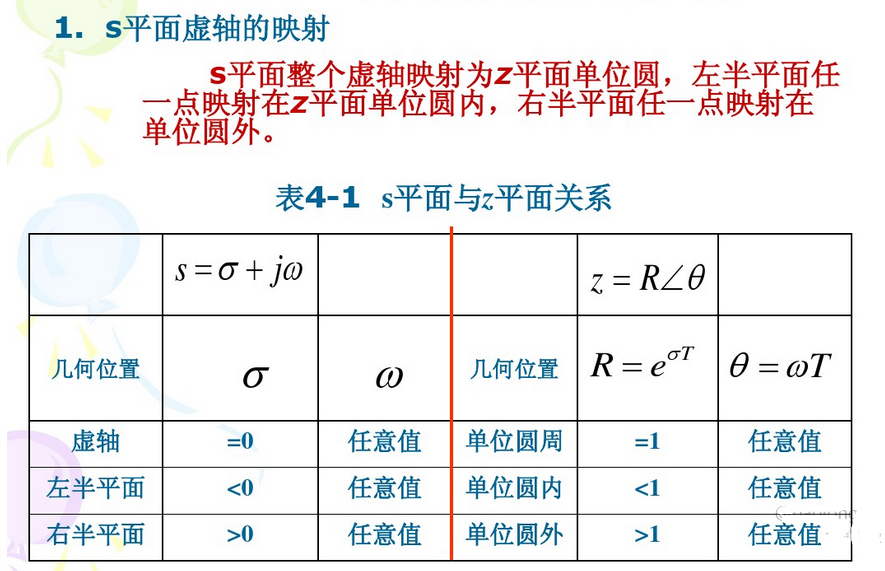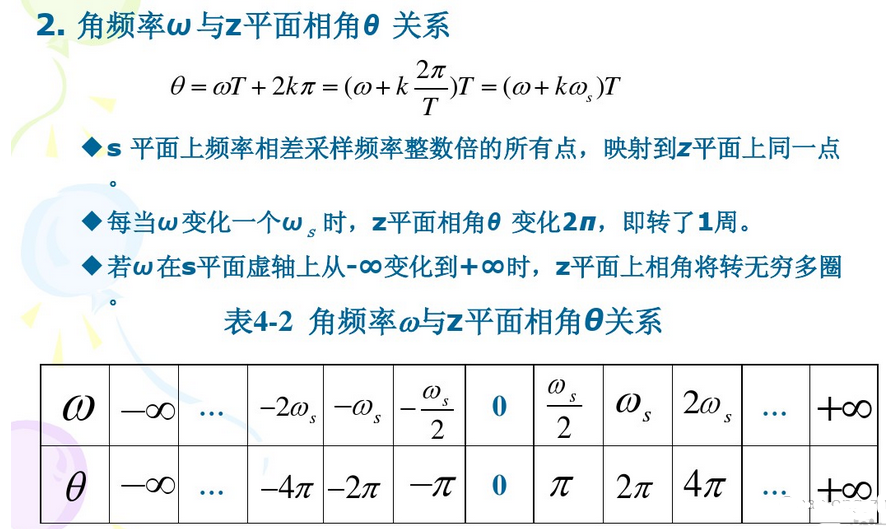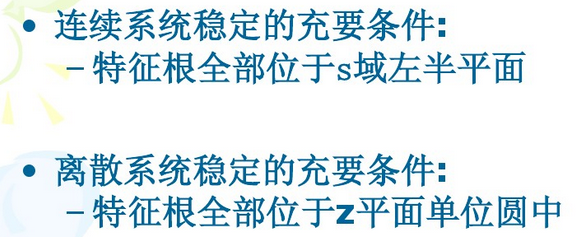展开全文• 奥本海姆版 信号与系统主要公式和内容摘要，对于学习信号与系统非常重要
• 信号与系统的简单总结1，总结了傅里叶变换的一些公式与使用MATLAB的实现，待续
• 信号与系统精华总结，非常详细的公式总结，内容丰富以及合理。
• 内容包括了信号与系统这门课程的重要知识点，概念以及公式集总 很多的知识点的解说直接考题挂钩 可能也有疏忽吧，毕竟我不可能做完所有的题目 总而言之，还是很花了一点功夫 希望各位想学好这门课程的人都能够取得...
• 还是齐开悦博士的[视频](https://www.bilibili.com/video/av5868266/?p=20)，不过这次没看完就自己看着书总结了（还是觉得看书更加高效率）。
还是齐开悦博士的视频，不过这次没看完就自己看着书总结了（还是觉得看书更加高效率）。
重新提一下，一定要把课本的例题过一遍，因为例题有很详细的解析（孙国霞的书的话比较少资料，贫僧觉得还是看吴大正的比较好，至少课后习题有答案解析，这样可以多很多习题来练手。现在流的汗都是当初买错书脑子进的水。。。），而且做完之后可以看得出自己那一步想漏了或者想错了，所以无论如何都要过一遍。
首先复习一下前面讲的拉氏变换： 拉氏变换比傅里叶变换的适用范围更加广泛，但是还是被收敛域限制住了（而傅里叶的限制是被积函数绝对可积）。为了保证能够积分出答案，所以要对

σ

\sigma

有限制，这就是收敛域。

∫

−

∞

+

∞

f

(

t

)

e

−

σ

t

e

−

j

ω

t

d

t

\int_{-\infty}^{+\infty}f(t)e^{-\sigma t}e^{-\mathrm{j}\omega t}\mathrm{d}t

所以每次答题的时候一定要加上对应的收敛域，不然公式没意义（可能结果不成立）。 比较有用的是单边，因为可以用来算零状态和零输入。 要记清楚正负号对收敛域的影响（例如

e

−

a

t

u

(

t

)

→

1

s

+

a

e^{-at}\mathrm{u}(t) \rightarrow \frac{1}{s + a}

的定义域是

R

e

[

s

]

&gt;

−

a

Re[s] &gt; -a

，而

e

a

t

u

(

−

t

)

→

−

1

s

−

a

e^{at}\mathrm{u}(-t) \rightarrow -\frac{1}{s - a}

但是定义域方向是翻过来的

R

e

[

s

]

&lt;

+

a

Re[s] &lt; +a

。
基本性质中拉氏变换只比傅里叶变换多了初值和终值定理，其他的差不多。 一定要记住两阶的微分性质（通用的是

f

(

n

)

(

t

)

s

n

F

(

s

)

−

∑

m

=

0

n

−

1

s

n

−

1

−

m

f

(

m

)

(

0

−

)

f^{(n)}(t)s^nF(s) - \sum_{m = 0}^{n-1}s^{n - 1 - m}f^{(m)}(0_-)

两阶就是

f

(

2

)

(

t

)

=

s

2

F

(

s

)

−

s

1

f

(

0

)

(

0

−

)

−

s

0

f

(

1

)

(

0

−

)

f^{(2)}(t)=s^2F(s) - s^{1}f^{(0)}(0_-) - s^0f^{(1)}(0_-)

）。 熟练用拉氏反变换的部分分式展开法（记得注意收敛域，如果题目没有给收敛域的话就要另外讨论）。 熟练用拉氏变换来分析电路（记得各种元件对应的s域模型，记串联的基本上就可以了）。 周期信号的拉氏变换是

F

(

s

)

=

F

1

(

s

)

1

1

−

e

−

s

T

R

e

[

s

]

&gt;

0

F(s) = F_1(s)\frac{1}{1-e^{-sT}} \quad Re[s] &gt; 0

，

F

1

(

s

)

F_1(s)

就是主周期的信号对应的拉氏变换结果。主要用在反变换，遇到要逆变换的函数的分母含有

1

−

e

−

s

T

1-e^{-sT}

的时候就可以直接推出对应的原函数（周期为T的周期信号）。

一些定义
一定要记住定义，这样遇到问题的时候（例如欸欸欸为什么答案是这样，以及啊啊啊不记得公式了啊）就可以回到定义/原理来推导（推导过程也可以帮助记忆，理解其实就是记住原理和各个推导出来的公式之间的关系，理解越深就离定义越近）。

·连续信号

x

(

t

)

x(t)

经过均匀冲激序列进行理想抽象后可以得到抽样信号

x

s

(

t

)

x_s(t)

：

x

s

(

t

)

=

x

(

t

)

σ

T

s

(

t

)

=

x

(

t

)

∑

n

=

−

∞

∞

σ

(

t

−

n

T

s

)

=

∑

n

=

−

∞

∞

x

(

n

T

s

)

σ

(

t

−

n

T

s

)

x_s(t) = x(t) \sigma_{Ts}(t) = x(t)\sum_{n = -\infty}^{\infty}\sigma(t - nT_s) = \sum_{n = - \infty}^{\infty}x(nT_s)\sigma(t - nT_s)

对等式两边同时取拉普拉斯变换：

X

s

(

s

)

=

∫

−

∞

∞

x

s

(

t

)

e

−

s

t

d

t

=

∫

−

∞

∞

e

−

s

t

∑

n

=

−

∞

∞

x

(

n

T

s

)

σ

(

t

−

T

s

)

d

t

X_s(s) = \int_{-\infty}^{\infty}x_s(t)\mathrm{e}^{-st}\mathrm{d}t = \int_{-\infty}^{\infty}\mathrm{e}^{-st}\sum_{n = -\infty}^{\infty}x(nT_s)\sigma(t - T_s)\mathrm{d}t

上面这个式子要注意积分的对象是

t

t

，不是

T

s

T_s

，所以

x

(

n

T

s

)

x(nT_s)

不受积分影响（相当于是常数了）。然后再把积分和求和顺序调换，用冲激函数的抽样性质就可以得到下面这个公式：

X

s

(

s

)

=

∑

n

=

−

∞

∞

x

(

n

T

s

)

e

−

s

n

T

s

X_s(s) = \sum_{n = -\infty}^{\infty}x(nT_s)\mathrm{e}^{-snT_s}

然后就像在拉普拉斯变换里面干的那样，用一个新的变量代替掉

x

x

以外的东西：

z

=

e

s

T

s

z = \mathrm{e}^{sT_s}

就可以得到

X

(

z

)

=

∑

n

=

−

∞

∞

x

(

n

T

s

)

z

−

n

X(z) = \sum_{n = -\infty}^{\infty}x(nT_s)z^{-n}

这就是z变换，通常抽样的方式是均匀抽样，所以可以忽略掉

T

s

T_s

：

X

(

z

)

∑

n

=

−

∞

∞

x

(

n

)

z

−

n

X(z) \sum_{n = -\infty}^{\infty}x(n)z^{-n}

这里的定义用到了冲激信号的采样特性，同时要注意的是

σ

(

t

)

\sigma(t)

无论在哪种变换里面变换的结果都是

1

1

。 上面那种公式其实是双边z变换的定义式，如果是单边的话（现实中大部分都是单边的，所以这个定义式才有用）：

X

(

z

)

=

∑

n

=

0

∞

x

(

n

)

z

−

n

X(z) = \sum_{n = 0}^{\infty}x(n)z^{-n}

因为定义的过程中用到了拉普拉斯变换，所以正变换和反变换可以表示成：

X

(

z

)

=

L

[

x

(

n

)

]

x

(

n

)

=

L

−

1

[

X

(

z

)

]

X(z) = L[x(n)]\\ x(n) = L^{-1}[X(z)]

大写的X就是变换之后的像函数，小写的x对应的是原函数。
所以这里也可以看出来某些拉普拉斯变换里面出现的解题方法也可以用在z变换这里，例如部分分式展开法（但是要对原函数

x

(

n

)

x(n)

处理一下，下面会提到）。

z

z

平面与

s

s

平面的映射关系
从上面定义的公式里面可以看出来

z

z

和

s

s

之间有这样的关系：

z

=

e

σ

T

s

s

=

1

T

s

l

n

z

z = \mathrm{e}^{\sigma T_s}\quad s = \frac{1}{T_s}\mathrm{ln}z

将

s

s

用直角坐标系来表示，

z

z

用极坐标系来进行表示，那么有

s

=

σ

+

j

ω

,

z

=

∣

z

∣

e

j

Ω

s = \sigma + \mathrm{j}\omega, z = |z|\mathrm{e}^{\mathrm{j}\Omega}

，那么：

z

=

e

s

T

s

=

e

(

σ

+

j

ω

)

T

s

z = \mathrm{e}^{sT_s} = \mathrm{e}^{(\sigma + \mathrm{j}\omega)T_s}

因为

z

=

∣

z

∣

e

j

Ω

z = |z|\mathrm{e}^{\mathrm{j}\Omega}

，所以：

∣

z

∣

=

e

σ

T

s

Ω

=

ω

T

s

=

ω

f

s

|z| = \mathrm{e}^{\sigma T_s} \\ \Omega = \omega T_s = \frac{\omega}{f_s}

上面表明了数字角频率

Ω

\Omega

是模拟角频率

ω

\omega

关于采样频率

f

s

f_s

的归一化频率。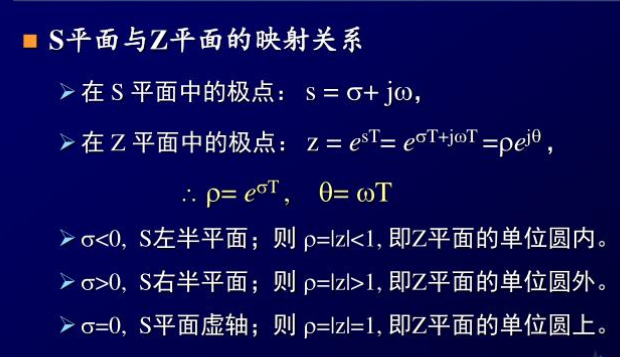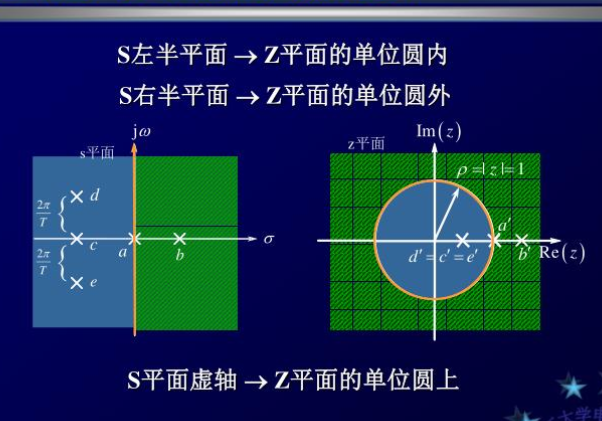上面这两张图片来自这里。
这里的映射关系在后面学习《数字信号处理》的时候会用到，不过现在只需要了解下就行。
收敛域
每一个

X

(

z

)

X(z)

可能会对应多个

x

(

n

)

x(n)

，所以需要指明收敛域来确定对应的是哪一个

x

(

n

)

x(n)

，且只有指明了收敛域，才可以确保

X

(

z

)

X(z)

收敛，使得公式有意义。
收敛域的特性：
收敛域是相通的，收敛域内不能包括任何极点，收敛域以极点为边界时限序列的收敛是整个

z

z

平面，但是

z

=

0

z=0

或

z

=

∞

z=\infty

可能除外因果序列的收敛域是以像函数

X

(

z

)

X(z)

的最大极点的模为半径的圆外区域非因果序列的收敛域是以像函数

X

(

z

)

X(z)

的最小极点的模为半径的圆内区域
常用公式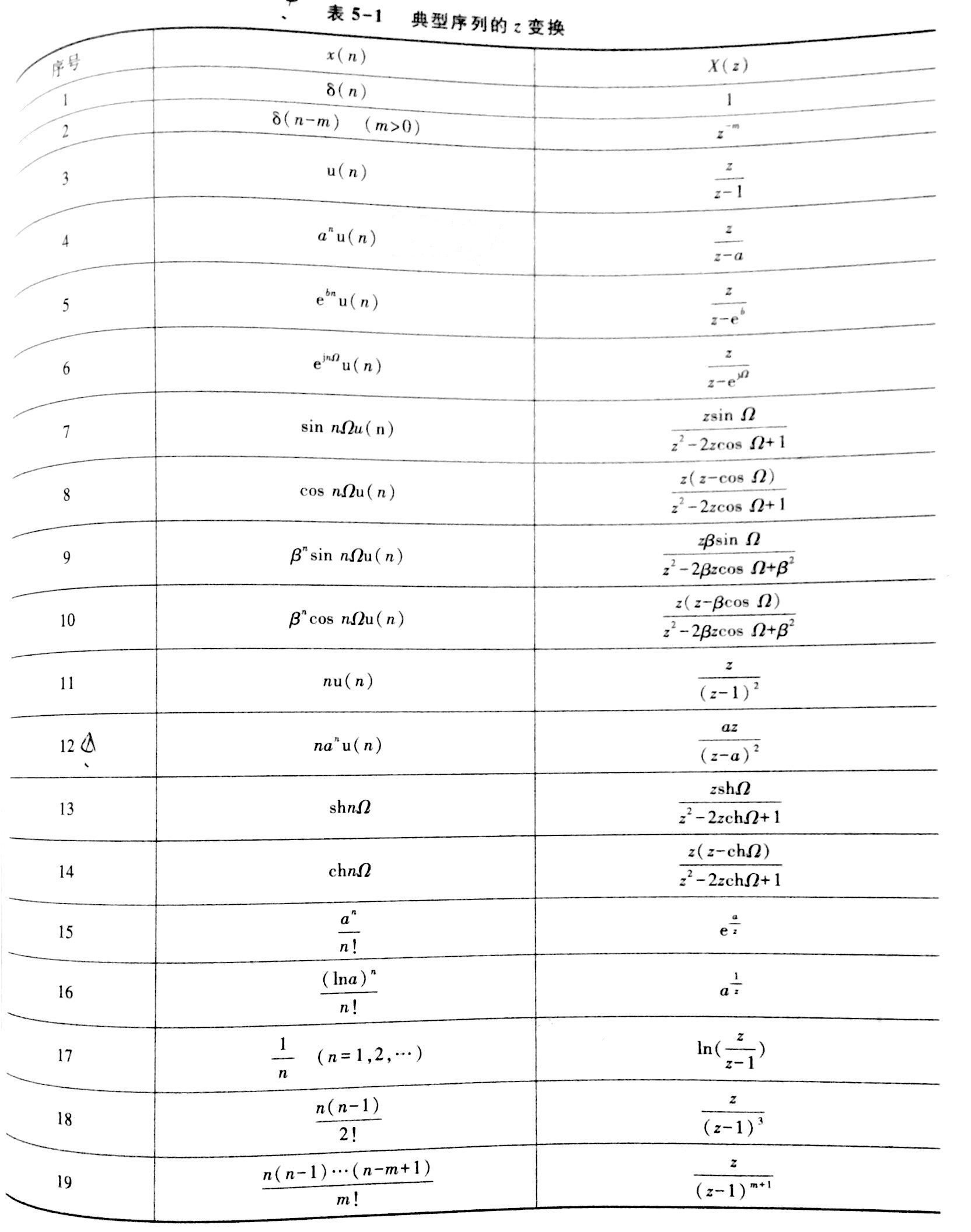上图来自孙国霞老师的《信号与系统》。
常用性质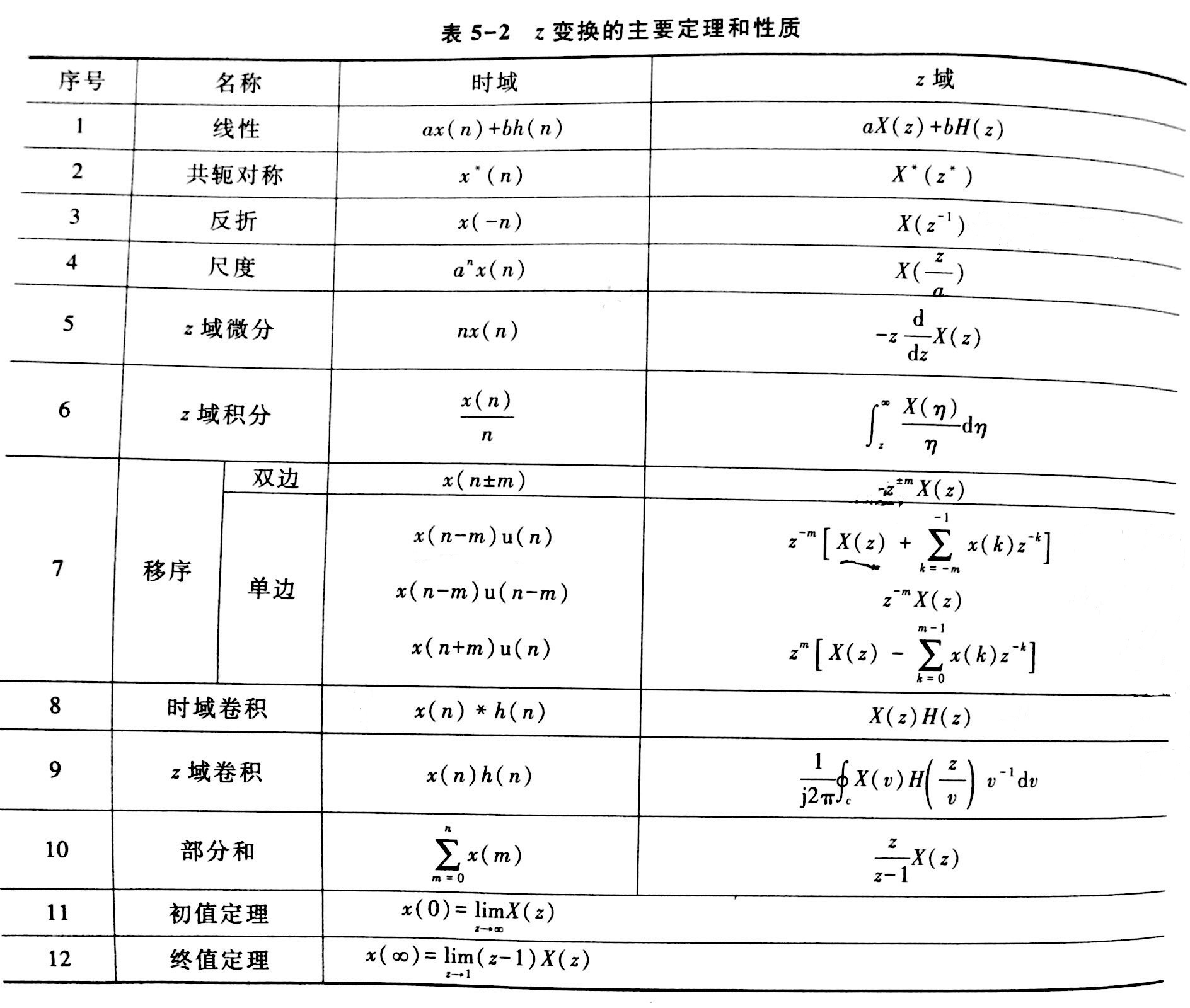上图来自孙国霞老师的《信号与系统》。
z反变换
反变换公式定义：

x

(

n

)

=

1

2

π

j

∮

c

X

(

z

)

z

n

−

1

d

z

x(n) = \frac{1}{2\pi \mathrm{j}}\oint_{c}X(z)z^{n-1}\mathrm{d}z

除非万不得已，通常不用定义式，而是：1. 长除法；2. 部分分式法（重点）
长除法
长除法很简单，直接给个例子自己体会：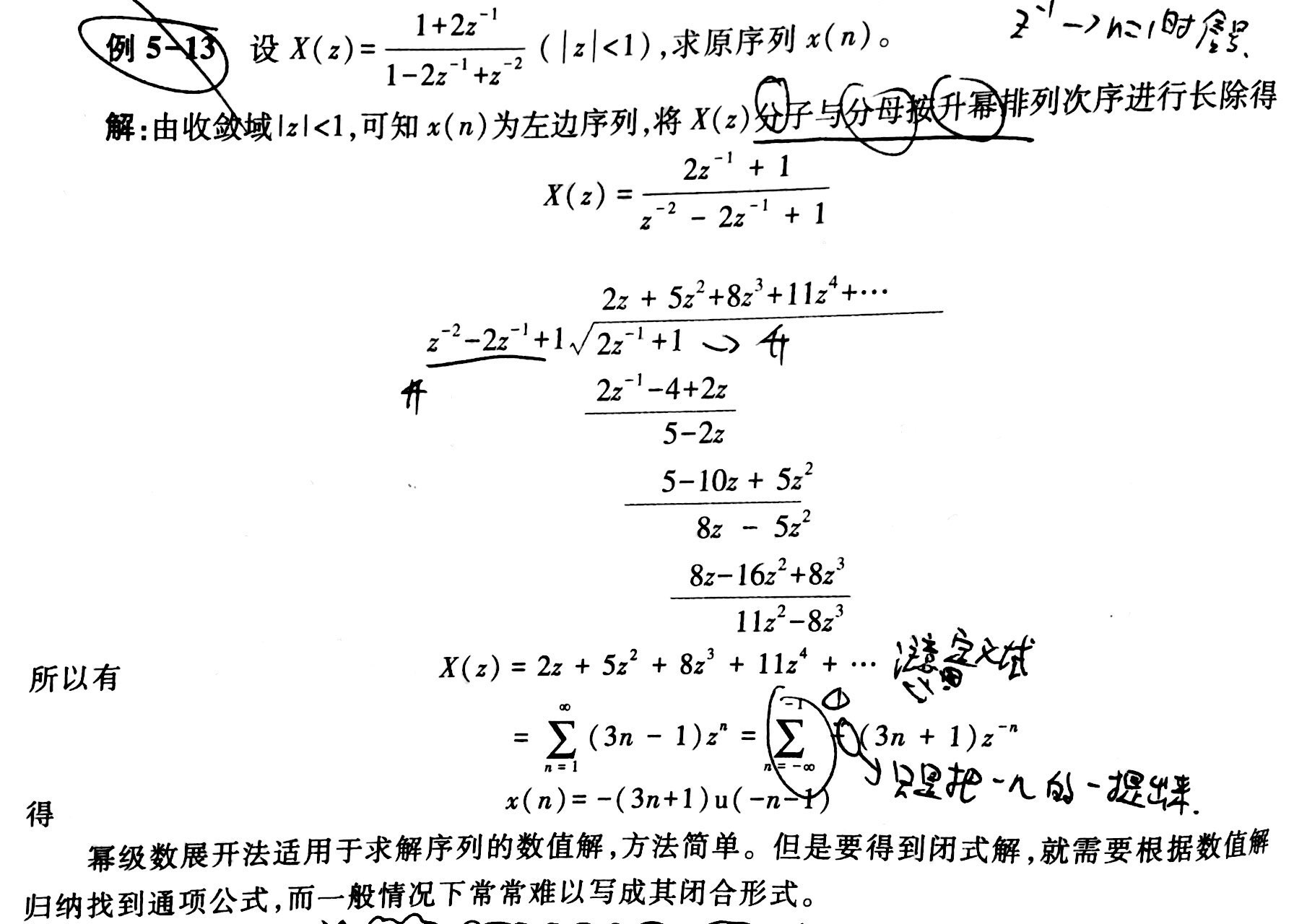部分分式法
部分分式法直接参考之前拉普拉斯变换里面的部分分式法，都是一样的，所以这里只给个例子。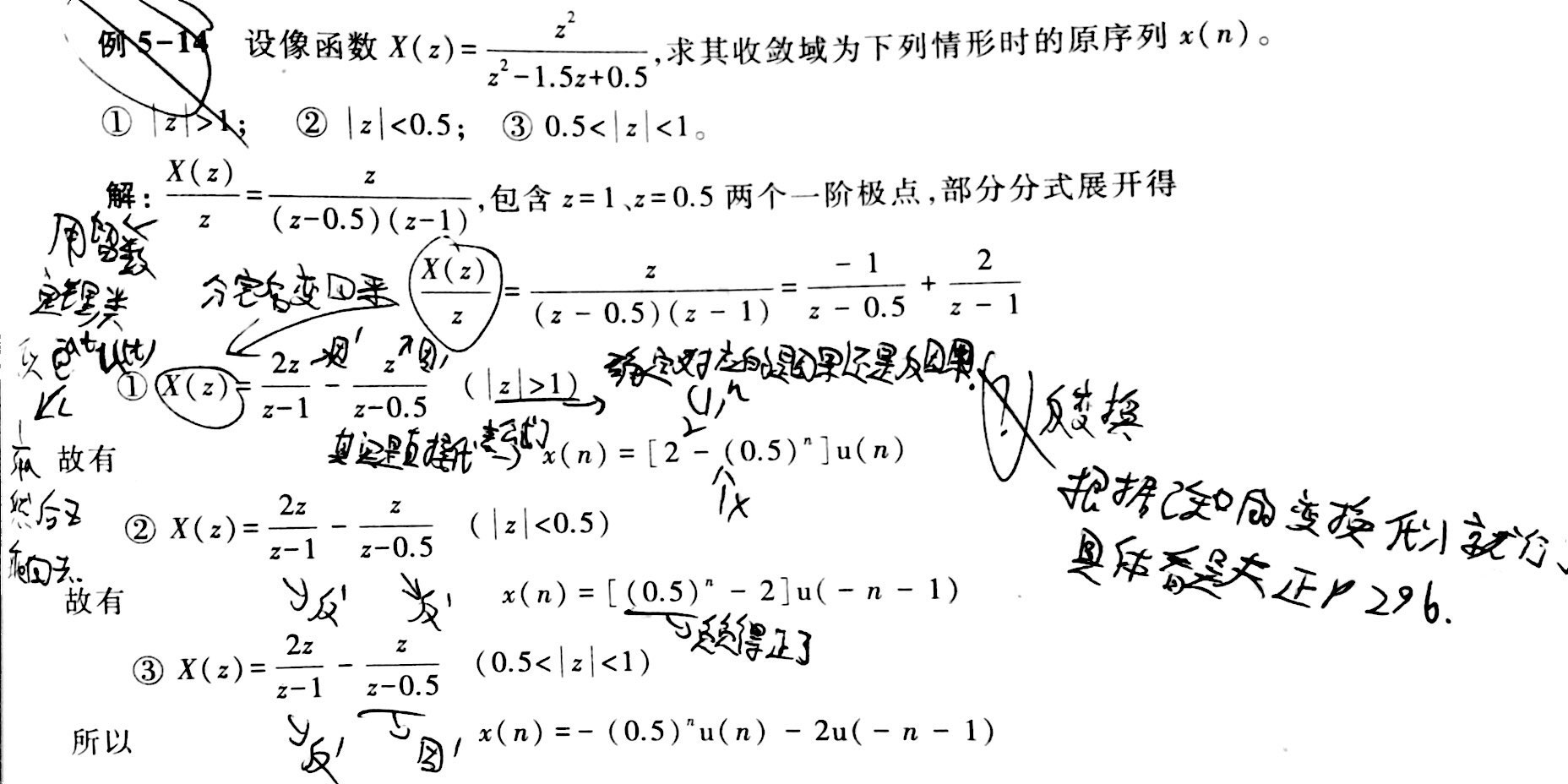差分方程的变换解
其实这里和拉普拉斯解微分方程是差不多的，只是比较不同的是有时需要用到迭代法（即对原方程中

y

(

n

)

y(n)

的

n

n

代入需要的数字来求出对应时刻系统的输出）。这里给个例子（没有用到迭代法，因为题目已经给出了所有需要用到的

y

(

n

)

y(n)

：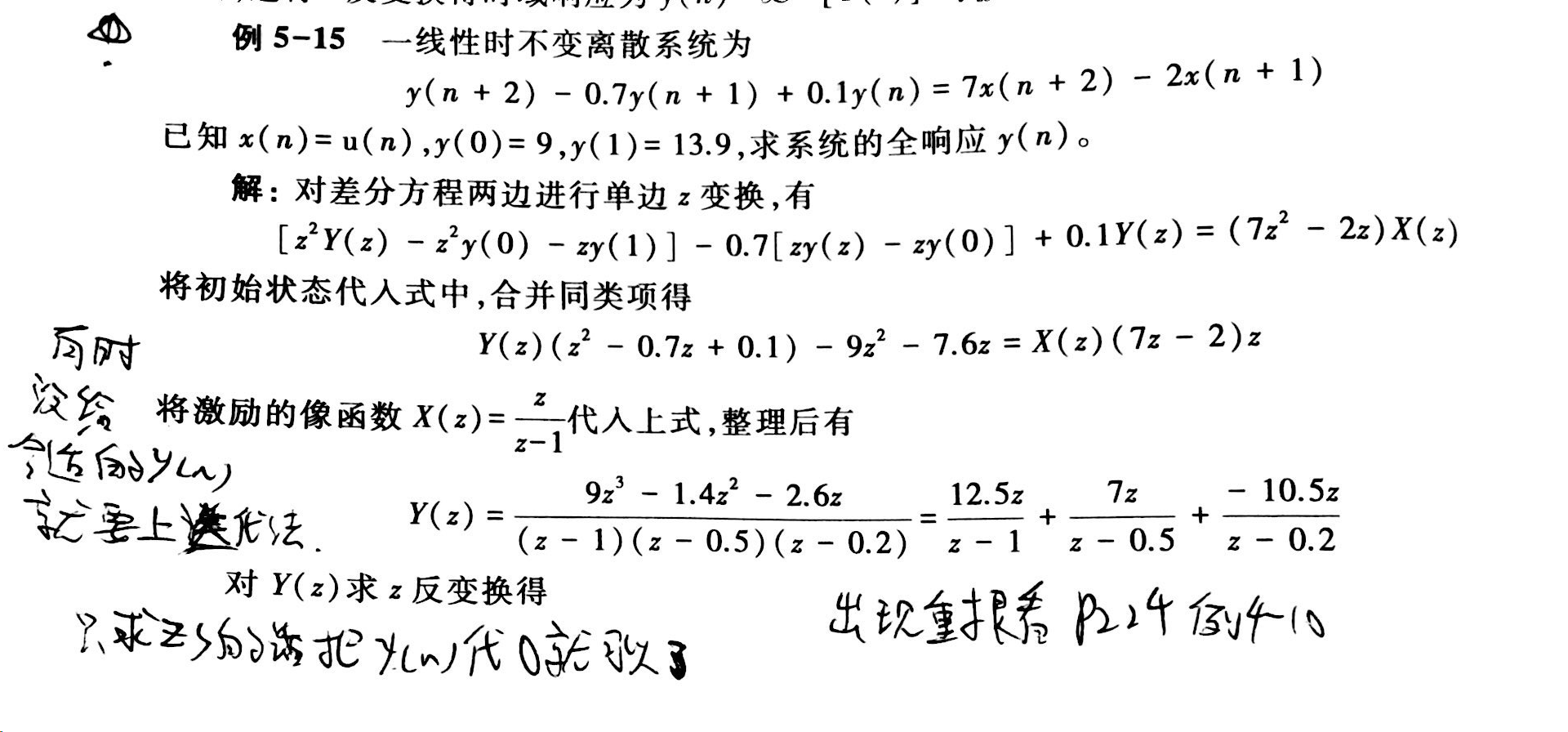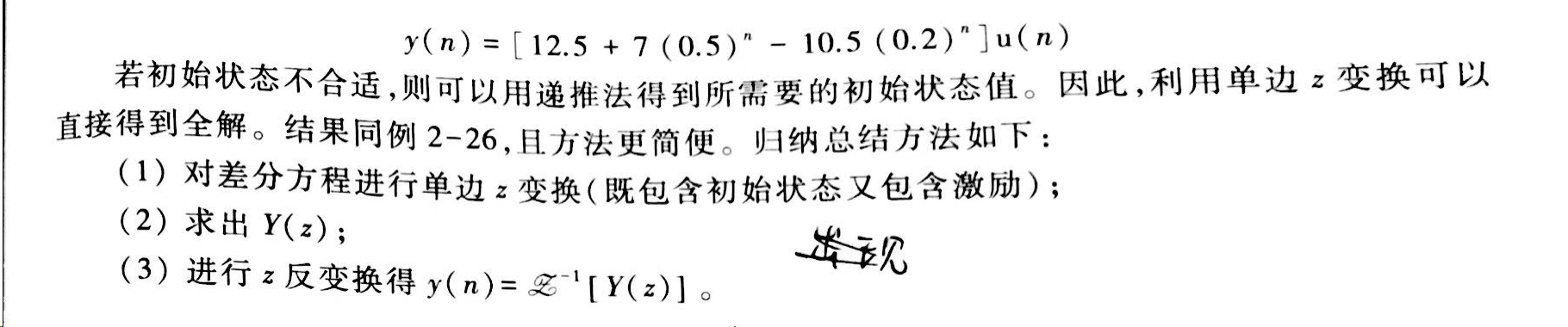系统函数和冲激响应

h

(

n

)

h(n)

和之前拉普拉斯、傅里叶变换的系统函数定义是一样的。z变换中零状态响应等于激励与系统冲激响应（即输入为

δ

(

n

)

\delta(n)

，冲激函数，时系统的输出）的卷积和，

y

z

s

(

n

)

=

x

(

n

)

∗

h

(

n

)

y_{zs}(n) = x(n) * h(n)

，

Y

z

s

(

z

)

=

X

(

z

)

H

(

z

)

Y_{zs}(z) = X(z)H(z)

，这里的

H

(

z

)

H(z)

就是离散系统的系统函数，定义为z域中零状态响应与激励的像函数的比值，

H

(

z

)

=

Y

z

s

(

z

)

X

(

z

)

H(z) = \frac{Y_{zs}(z)}{X(z)}

。注意，

H

(

z

)

H(z)

只与系统特性相关，只要系统不变，系统函数就不会变（这点上3个变换都是这样）。
此外，解题的时候记得写这句话：在零状态条件下，对差分方程两边取单边z变换。不然可能会因为不严谨而失分，因为在使用系统函数的时候就已经架设了初始条件为0（即处于零状态）且激励函数为因果序列（看公式表，如果不是因果序列z变换之后的结果会有“小尾巴”）。
此外，因为系统本身的特性决定了系统函数，所以可以通过系统函数可以判断系统的特性：参考资料
《信号与系统》孙国霞（基本上这篇博文里面的公式都是从这里来的，但就算是这样，贫僧还是要说，用吴大正的吧。。。目前这本书还没有习题解析之类的辅导书，所以自学用的话会比较麻烦，网上又不一定能找得到答案） 《信号与线性系统分析（第 4 版）》吴大正（贫僧其实是拿这本书当参考书来用了，看上面那一本书看不懂的时候就会看下面这一本，因为上面那一本总结的挺好，但是不适合初学者。贫僧觉得想要找到比较详细的解析的话还是看吴大正这本比较好） 齐开悦博士的视频（超有用。。。然而并不能代替刷题，如果是要考试的话该刷的还要刷，如果只是自学的话还是挺推荐的） §4 Z 变换与拉氏变换的关系
展开全文• 信号与系统课程中常用的定理公式,还有解题方法的介绍,总结得简明扼要.非常适合考试.
• 目录第一章 绪论信号与系统信号的概念系统的概念信号的描述分类信号的描述信号的分类系统的描述分类系统的分类连续系统的描述离散系统的描述系统的性质线性时不变性因果性稳定性即时性LTI系统分析方法概述第二章...


目录
第一章 绪论信号与系统信号的概念系统的概念
信号的描述与分类信号的描述信号的分类
系统的描述与分类系统的分类连续系统的描述离散系统的描述
系统的性质线性时不变性因果性稳定性即时性
LTI系统分析方法概述
第二章 连续时间信号与系统的时域分析常用信号及信号的基本运算常用信号(需数熟练掌握)信号的基本运算
阶跃信号和冲激信号阶跃信号冲激信号
零输入响应(零状态响应)阶跃响应和冲激响应阶跃响应和冲激响应冲激响应的求解
卷积积分信号时域分解与卷积卷积的图解卷积积分的性质卷积代数奇异函数的卷积特性卷积的微积分性质卷积的时移特性
连续系统的时域分析

第三章 连续系统的频域分析周期信号的傅里叶级数非周期信号的频谱——傅里叶变换傅里叶变换的性质连续时间系统的频域分析
第四章 连续系统的s域分析拉普拉斯变换从傅里叶变换到拉普拉斯变换收敛域(单边)拉普拉斯变换
单边拉普拉斯变换的性质拉普拉斯变换逆变换连续时间系统的复频域分析微分方程的变换解系统函数
系统特性与系统函数的关系
第五章 离散系统的时域分析离散信号与离散系统离散时间信号的表示典型离散信号离散信号的基本运算离散系统响应的求解方法
离散系统的零输入响应单位样值响应和阶跃响应离散系统的零状态响应卷积和卷积的性质卷积的计算

第六章 离散系统z域分析z 变换从拉普拉斯变换到z变换收敛域
z 变换的性质逆z变换离散系统的 z 域分析差分方程的变换解系统函数系统因果性和稳定性

解题方法及注意事项线性判断方法常见类型
因果性的判定方法时变与时不变判断冲激响应的求解卷积相关奇异信号卷积化简
傅里叶相关利用傅里叶变换的性质要注意:傅里叶逆变换
门信号和三角信号门信号三角信号
希尔伯特变换时域-频域-S域-Z域时域与频域之间的频谱关系
计算问题频率响应的滤波特性部分分式展开F(z)和F(s)做部分分式展开的区别展开技巧
系统稳定性判断奈奎斯特
响应的初始值求解理解零输入响应&零状态响应求解连续系统零输入响应零状态响应
离散系统零输入响应零状态响应
微分方程和差分方程求解注意事项
信号流图系统框图连续系统离散系统求解注意
阶跃响应求解拉普拉斯初值定理和终值定理初值定理的使用条件终值定理的使用条件初值定理终值定理
(简易)一元三次方程拆分/求根方法拆解步骤重根情况判断是否存在+1,-1的根
方法失效的情况

第一章 绪论
信号与系统
信号的概念
信号是消息的表现形式,消息则是信号的具体内容.带有信息的随时间变化的物理量.
系统的概念
系统可看做是对洗脑进行存储、转换、传输、和处理的物理装置.若干相互作用和相互依赖的事物组合而成的具有特定功能的整体.
信号的描述与分类
信号的描述
描述信号的基本方法是建立信号的数学模型,即写出信号的数学表达式.一般地,描述信号的数学表达式都以时间为变量,即数学表达式都是时间的函数,绘出函数的图像称为信号的波形.信号的描述可分为两种:函数表达式和波形.
信号的分类
确定信号与随机信号连续时间信号离散时间信号周期信号与非周期信号能量信号与功率信号实信号与复信号一维信号与多维信号
系统的描述与分类
系统的分类
连续系统:系统的输入和输出信号均为连续信号离散系统:系统的输入和输出信号均为离散信号混合系统:系统的输入信号是连续信号,输出信号是离散信号.或者反之.连续时间系统与离散时间系统即时系统与动态系统集总参数系统与分布参数系统线性系统与非线性系统时变系统与时不变系统
连续系统的描述
连续系统:系统的输入和输出信号均为连续信号
离散系统的描述
离散系统:系统的输入和输出信号均为离散信号
系统的性质
线性、时不变性、因果性、稳定性、即时性
线性
满足分解性、齐次性、可加性
分解性:全响应y(t)可分解为零输入响应yzi(t)和零状态响应yzs(t).

y(t) = yzi(t) + yzs(t)

齐次性:激励f1(t)增大α倍时,其响应也增大α倍.

f(t) —> y(t) αf(t) —> αy(t)

可加性:系统对于激励f1(t)与f2(t)之和的响应等于各个激励单独作用所引起响应之和

f1(t) —> y1(t) f2(t) —> y2(t) f1(t) + f2(t) = y(t) = y1(t) + y2(t)

时不变性
激励为f(t)时产生的零状态响应为yzs(t),若激励延迟一定时间t0接入,即f(t-t0)时,其响应也延迟t0,为yzs(t-t0).反之为时变.
因果性
系统在任意时刻的输出值仅取决于现在或过去的输入,而与将来的输入无关.
稳定性
输入有界(幅值为有限制),零状态响应也有界.|f(t)|<∞,其零状态|yzs(t)|<∞,则称该系统是稳定的.
即时性
即时系统:系统在任意时刻的响应,仅取决于该时刻的激励,而与它过去的工作状态无关,则称为即时系统(无记忆系统).动态系统:系统在任意时刻的响应不仅取决于该时刻的激励,而且与它过去的工作状态有关,则称为动态系统(记忆系统).
LTI系统分析方法概述
系统分析的主要任务是通过求解给定系统在已知激励下的响应,分析系统具有的特性和功能.系统分析就建立系统的数学模型,然后用数学方法进行求解,对所得结果进行物理解释,并赋予物理含义.系统的描述方法 – 微分(差分)方程 – 输入-输出(外部法) – 状态变量法(内部法)系统的分析方法 – 时域法 – 变换域法 — 频域分析 — 复(s)频域分析 — z域分析
第二章 连续时间信号与系统的时域分析
常用信号及信号的基本运算
常用信号(需数熟练掌握)
门信号 正三角脉冲 冲激信号 直流信号 阶跃信号 单边指数信号 双边指数信号 余弦信号 正弦型号 有始余弦信号 有始正弦信号
信号的基本运算
加法 减法 乘法
阶跃信号和冲激信号
阶跃信号
U(t) t > 0 时为1 t < 0 时为0
冲激信号
δ(t) t = 0 为∞,其余为0
零输入响应
系统输入为零,仅系统本身产生的响应
(零状态响应)阶跃响应和冲激响应
系统状态为零,仅输入激励产生的响应
阶跃响应和冲激响应
(冲激响应) 当激励信号为单位冲激函数δ(t)时,系统产生的零状态响应称为单位冲激响应 (阶跃响应) 当激励信号为单位阶跃函数u(t)时,系统产生的零状态响应称为单位阶跃响应
冲激响应的求解
经典解法
求微分方程的齐次解 y(t)≥0h(t)=y(t)u(t)分别求h’(t),h’’(t),…h(n)(t).求导过程利用f(t)δ(t)=f(0)δ(t)的性质化简将h’(t),h’’(t),…h(n)(t)等带入微分方程中,并令输入f(x)=δ(t)令式子两端δ(t),δ’(t),…δ(n)(t)的系数相等,得到一组方程组求解该方程组得到微分方程齐次解中的待定系数将待定系数带入h(t)中即求得冲激响应h(t)
已知阶跃响应求冲激响应 对阶跃响应求导即可已知系统函数H(S),H(Z) 对系统函数进行逆变换即可已知G(S),G(Z) 通过H(S)=G(S)/L[U(t)]求出系统函数H(S),然后再逆变换即可 通过H(Z)=G(Z)/L[U(t)]求出系统函数H(Z),然后再逆变换即可
卷积积分
信号时域分解与卷积
将一个信号分解成一个个的单位冲激信号 将每个单位冲激信号依次进入系统等于各个单位冲激信号的响应 将单位冲激响应全部叠加即零状态响应
卷积的图解
反折 从无穷远的地方到与信号重叠的前一刻均为0 从与信号重叠部分开始求面积,若为分段信号则要再分区间讨论 当反折信号开始不再重叠的时候,再次分段计算求面积
卷积积分的性质
结合律 分配律 交换律 微分 积分 微积分 延迟
卷积代数
奇异函数的卷积特性
卷积的微积分性质
卷积的时移特性
连续系统的时域分析
第三章 连续系统的频域分析
周期信号的傅里叶级数
非周期信号的频谱——傅里叶变换
傅里叶变换的性质
连续时间系统的频域分析
第四章 连续系统的s域分析
拉普拉斯变换
从傅里叶变换到拉普拉斯变换
收敛域
(单边)拉普拉斯变换
单边拉普拉斯变换的性质
拉普拉斯变换逆变换
连续时间系统的复频域分析
微分方程的变换解
系统函数
系统特性与系统函数的关系
第五章 离散系统的时域分析
离散信号与离散系统
离散时间信号的表示
典型离散信号
离散信号的基本运算
离散系统响应的求解方法
离散系统的零输入响应
单位样值响应和阶跃响应
离散系统的零状态响应
卷积和
卷积的性质
卷积的计算
第六章 离散系统z域分析
z 变换
从拉普拉斯变换到z变换
收敛域
z 变换的性质
逆z变换
离散系统的 z 域分析
差分方程的变换解
系统函数
系统因果性和稳定性
解题方法及注意事项
线性判断方法
1.设f1(t)→y1(t),f2(t)→y2(t) 2.令f3(t)=af1(t)+bf2(t) 3.根据f3(t)求出y3(t) 4.判断y3(t)是否等于ay1(t)+by2(t),若相等为线性,反之为非线性.
常见类型
y(t)性质[f(t)]n非线性(at-t0)f(t)线性sin[f(t)]非线性∫t-∞f(x)dx线性af’(t)线性f(at)线性
因果性的判定方法
分别令t=0和t=1,则有y(0)和y(1),此时若式中只有f(0)或f(1)或f(x)x<0或x<1, 则表明响应取决于当前或之前的激励,故因果. 若f(x)中的x>0或x>1则表明响应取决于未来的激励,故非因果.
时变与时不变判断
1.将式子写成左边仅保留y(t)的形式(即使有y(n)(t)也移到右边). 2.将f(t)进行延时成f(t-t0),此时对应的输出为yd(t),包括y(n)(t). 3.将原式y(t)进行延迟成y(t-t0),此时右端所有的t都换成(t-t0). 4.比较yd(t)和y(t-t0),若相等则时不变,反之时变.
冲激响应的求解
经典解法
求微分方程的齐次解 y(t)≥0h(t)=y(t)u(t)分别求h’(t),h’’(t),…h(n)(t).求导过程利用f(t)δ(t)=f(0)δ(t)的性质化简将h’(t),h’’(t),…h(n)(t)等带入微分方程中,并令输入f(x)=δ(t)令式子两端δ(t),δ’(t),…δ(n)(t)的系数相等,得到一组方程组求解该方程组得到微分方程齐次解中的待定系数将待定系数带入h(t)中即求得冲激响应h(t)
已知阶跃响应求冲激响应 对阶跃响应求导即可.已知系统函数H(S),H(Z) 对系统函数进行逆变换即可.已知G(S),G(Z) 通过H(S)=G(S)/L[U(t)]求出系统函数H(S),然后再逆变换即可. 通过H(Z)=G(Z)/L[U(t)]求出系统函数H(Z),然后再逆变换即可.
卷积相关
当两个带有U(t)的信号进行卷积时,积分区域直接写成

∫

0

t

\int_0^t

,计算结果直接加上U(t).当一个带U(t)和一个带U(t-t0)的信号进行卷积时,积分区域直接写成

∫

0

t

−

t

0

\int_0^{t-t_0}

,结果直接加上U(t-t0).两个带常系数的信号卷积,系数部分直接相乘后放到最外边,再进行卷积.卷积的作用是求解零状态响应.
奇异信号卷积化简

δ

(

t

)

∗

δ

(

t

)

=

δ

(

t

)

\delta(t)*\delta(t)=\delta(t)

δ

(

t

)

∗

δ

(

t

−

n

)

=

δ

(

t

−

n

)

\delta(t)*\delta(t-n)=\delta(t-n)

δ

(

t

)

∗

U

(

t

)

=

U

(

t

)

\delta(t)*U(t)=U(t)

δ

(

t

)

∗

δ

(

t

−

1

)

∗

δ

(

t

−

1

)

=

δ

(

t

−

2

)

\delta(t)*\delta(t-1)*\delta(t-1)=\delta(t-2)

U

(

t

)

∗

U

(

t

)

=

t

U

(

t

)

U(t)*U(t)=tU(t)

δ

(

t

−

n

)

∗

U

(

t

)

=

U

(

t

−

n

)

\delta(t-n)*U(t)=U(t-n)

U

(

t

)

∗

[

−

δ

(

t

)

]

=

−

[

U

(

t

)

∗

δ

(

t

)

]

=

−

U

(

t

)

U(t)*[-\delta(t)]=-[U(t)*\delta(t)]=-U(t)

傅里叶相关
傅里叶变换得频移性质是在时域乘以一个旋转因子而不是指数信号,而公式

e

−

a

t

U

(

t

)

⟷

1

j

w

+

a

e^{-at}U(t)\longleftrightarrow\frac{1}{jw+a}

是指数信号的傅里叶变换,不是频移,若遇到

e

−

a

t

U

(

t

−

t

0

)

e^{-at}U(t-t_0)

求傅里叶变换一般用定义计算.对U(t)做傅里叶变换得频移、时移等不能直接在

U

(

t

)

⟷

π

δ

(

w

)

+

1

j

w

U(t)\longleftrightarrow\pi\delta(w)+\frac{1}{jw}

的基础上进行,因为

U

(

t

)

=

1

2

+

1

2

s

g

n

(

t

)

U(t)=\frac{1}{2}+\frac{1}{2}sgn(t)

而对U(t)进行时移、尺度变换等,时间是对sgn(t)做变换,若直接对

π

δ

(

t

)

+

1

j

w

\pi\delta(t)+\frac{1}{jw}

进行傅里叶变换必然出现问题. 综上遇到对U(t)使用傅里叶变换的性质时,要么拆分成

U

(

t

)

=

1

2

+

1

2

s

g

n

(

t

)

U(t)=\frac{1}{2}+\frac{1}{2}sgn(t)

再做变换,要么直接对

U

(

t

)

⟷

π

δ

(

w

)

+

1

j

w

U(t)\longleftrightarrow\pi\delta(w)+\frac{1}{jw}

中的

1

j

w

\frac{1}{jw}

做变换.

例:

U

(

1

2

t

−

1

)

U(\frac{1}{2}t-1)

错误计算: 1.

U

(

t

)

⟷

π

δ

(

w

)

+

1

j

w

U(t)\longleftrightarrow\pi\delta(w)+\frac{1}{jw}

2.

U

(

t

−

1

)

⟷

π

δ

(

w

)

e

−

j

0

+

1

j

w

e

−

j

w

U(t-1)\longleftrightarrow\pi\delta(w)e^{-j0}+\frac{1}{jw}e^{-jw}

3.

U

(

1

2

t

−

1

)

⟷

2

π

δ

(

2

w

)

e

−

j

0

+

1

j

w

e

−

2

j

w

U(\frac{1}{2}t-1)\longleftrightarrow2\pi\delta(2w)e^{-j0}+\frac{1}{jw}e^{-2jw}

正确计算: 1.

U

(

t

)

=

1

2

+

1

2

s

g

n

(

t

)

⟷

π

δ

(

w

)

+

1

j

w

U(t)=\frac{1}{2}+\frac{1}{2}sgn(t)\longleftrightarrow\pi\delta(w)+\frac{1}{jw}

2.

U

(

t

−

1

)

=

1

2

+

1

2

s

g

n

(

t

−

1

)

⟷

π

δ

(

w

)

+

1

j

w

e

−

j

w

U(t-1)=\frac{1}{2}+\frac{1}{2}sgn(t-1)\longleftrightarrow\pi\delta(w)+\frac{1}{jw}e^{-jw}

3.

U

(

1

2

t

−

1

)

=

1

2

+

1

2

s

g

n

(

1

2

t

−

1

)

⟷

π

δ

(

w

)

+

2

2

j

w

e

−

2

j

w

U(\frac{1}{2}t-1)=\frac{1}{2}+\frac{1}{2}sgn(\frac{1}{2}t-1)\longleftrightarrow\pi\delta(w)+\frac{2}{2jw}e^{-2jw}

若对带j的时域信号进行傅里叶变换,必然用到对称性质

F

(

j

t

)

⟷

2

π

f

(

−

w

)

F(jt)\longleftrightarrow2\pi f(-w)

但若是指数信号带j则表示频移.计算

e

±

a

U

(

∓

t

±

t

0

)

e^{\pm a} U(\mp t \pm t_0 )

时

e

±

a

U

(

∓

t

±

t

0

)

⟷

1

±

a

∓

j

w

e

±

t

0

(

±

a

∓

j

w

)

e^{\pm a} U(\mp t±t_0)\longleftrightarrow\frac{1}{\pm a\mp jw}e^{\pm t_0(\pm a\mp jw)}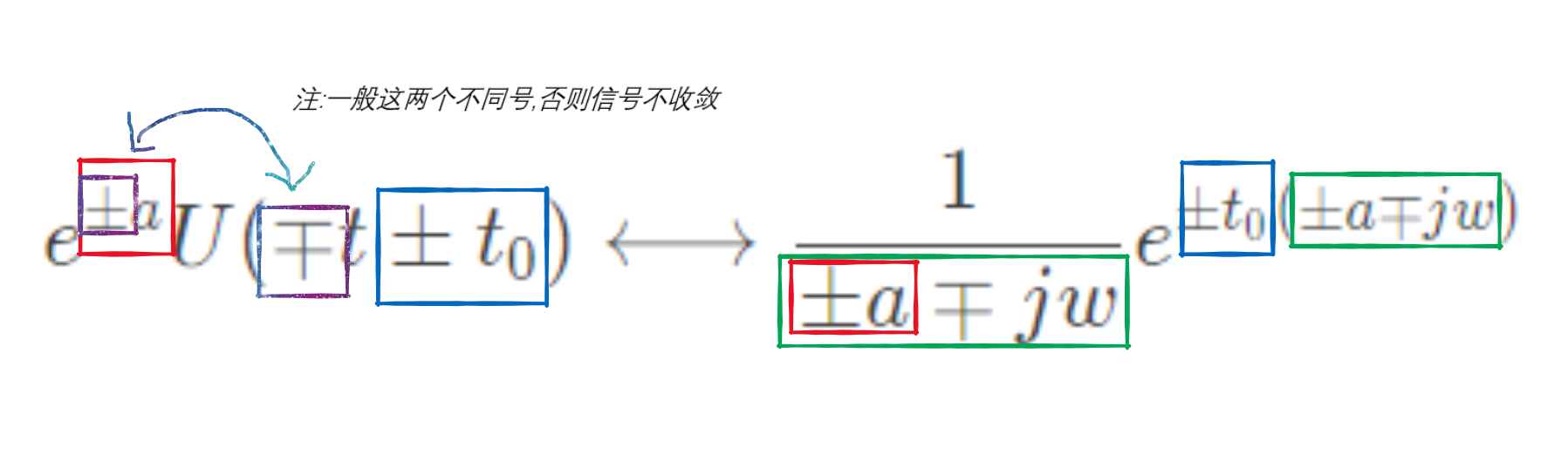利用傅里叶变换的性质要注意:

f

(

a

t

−

b

)

f(at-b)

:先进行时移再尺度

1.

F

(

j

w

)

e

−

b

j

w

1.F(jw)e^{-bjw}

2.

1

∣

a

∣

F

(

j

w

a

)

e

−

b

a

j

w

2.\frac{1}{|a|}F(j\frac{w}{a})e^{-\frac{b}{a}jw}

f

(

−

t

+

b

)

f(-t+b)

:先进行时移再反折

1.

F

(

j

w

)

e

b

j

w

1.F(jw)e^{bjw}

2.

F

(

−

j

w

)

e

−

j

w

2.F(-jw)e^{-jw}

f

(

a

−

b

t

)

f(a-bt)

:先进行时移再反折再尺度

1.

F

(

j

w

)

e

b

j

w

1.F(jw)e^{bjw}

2.

F

(

−

j

w

)

e

−

j

w

2.F(-jw)e^{-jw}

3.

1

∣

a

∣

F

(

j

w

a

)

e

−

b

a

j

w

3.\frac{1}{|a|}F(j\frac{w}{a})e^{-\frac{b}{a}jw}

−

t

f

(

−

t

)

-tf(-t)

不能看成

g

(

t

)

=

t

f

(

t

)

→

g

(

−

t

)

=

−

t

f

(

−

t

)

⟷

j

F

′

(

−

j

w

)

g(t)=tf(t)\rightarrow g(-t)=-tf(-t)\longleftrightarrow jF'(-jw)

应看成

f

(

−

t

)

⟷

F

(

−

j

w

)

f(-t)\longleftrightarrow F(-jw)

t

f

(

−

t

)

⟷

j

F

′

(

−

j

w

)

tf(-t)\longleftrightarrow jF'(-jw)

−

t

f

(

−

t

)

⟷

−

j

F

′

(

−

j

w

)

-tf(-t)\longleftrightarrow -jF'(-jw)

对

f

(

−

t

)

⟷

F

(

−

j

w

)

f(-t)\longleftrightarrow F(-jw)

做时移时,乘

e

−

j

w

t

e^{-jwt}

变成

e

j

w

t

e^{jwt}

或

e

j

w

t

e^{jwt}

变成

e

−

j

w

t

e^{-jwt}

利用时域相乘频域相卷积时,频域需乘一个

1

2

π

\frac{1}{2\pi}

傅里叶变换若化简成

f

[

a

(

t

−

b

)

]

f[a(t-b)]

的形式再做变换时要注意时移对应的部分不需要做尺度即

f

[

a

(

t

−

b

)

]

=

1

∣

a

∣

F

(

w

a

)

e

−

b

j

w

f[a(t-b)]=\frac{1}{|a|}F(\frac{w}{a})e^{-bjw}

在时域时域必须所有t进行改变例:

t

U

(

t

)

tU(t)

时移

(

t

−

1

)

U

(

t

−

1

)

(t-1)U(t-1)

傅里叶逆变换
做傅里叶变换逆变换时注意是否反折

例:

−

1

j

w

−

a

-\frac{1}{jw-a}

−

1

j

w

−

a

=

1

−

j

w

+

a

-\frac{1}{jw-a}=\frac{1}{-jw+a}

是经反折的,所以可以写成

1

j

w

−

a

\frac{1}{jw-a}

然后求逆变换得

e

a

t

U

(

t

)

e^{at}U(t)

再反折得

e

−

a

t

U

(

−

t

)

e^{-at}U(-t)

对于逆变换中常有

F

(

j

w

)

=

w

2

=

−

(

j

w

)

2

F(jw)=w^2=-(jw)^2

F

(

j

w

)

=

−

2

w

2

=

j

(

2

j

w

)

′

F(jw)=-\frac{2}{w^2}=j(\frac{2}{jw})'

F

(

j

w

)

=

1

(

j

w

+

a

)

2

=

j

(

1

j

w

+

a

)

′

F(jw)=\frac{1}{(jw+a)^2}=j(\frac{1}{jw+a})'

F

(

j

w

)

=

δ

(

w

)

⟷

1

2

π

F(jw)=\delta(w)\longleftrightarrow \frac{1}{2\pi}

F

(

j

w

)

=

[

U

(

w

)

−

U

(

w

−

w

0

)

]

=

g

w

0

(

w

−

w

0

2

)

F(jw)=[U(w)-U(w-w_0)]=g_{w_0}(w-\frac{w_0}{2})

f

(

−

w

)

=

1

2

π

F

(

j

t

)

f(-w)=\frac{1}{2\pi}F(jt)

f

(

w

)

=

1

2

π

F

(

−

j

t

)

f(w)=\frac{1}{2\pi}F(-jt)

门信号和三角信号
门信号

g

2

(

t

)

g_2(t)

表示宽为2的门信号,若进行尺度变换

g

2

(

a

t

)

g_2(at)

则可写成

g

a

2

(

t

)

g_\frac{a}{2}(t)

门信号做傅里叶变换之前尽量化简到以中心频率为

(

w

−

w

0

)

(w-w_0)

的点

例

g

2

(

w

−

w

0

)

+

g

2

(

w

+

w

0

)

g_2(w-w_0)+g_2(w+w_0)

因为存在频域,所以逆变换后会有旋转因子,不利于化简和计算故画出频域图像,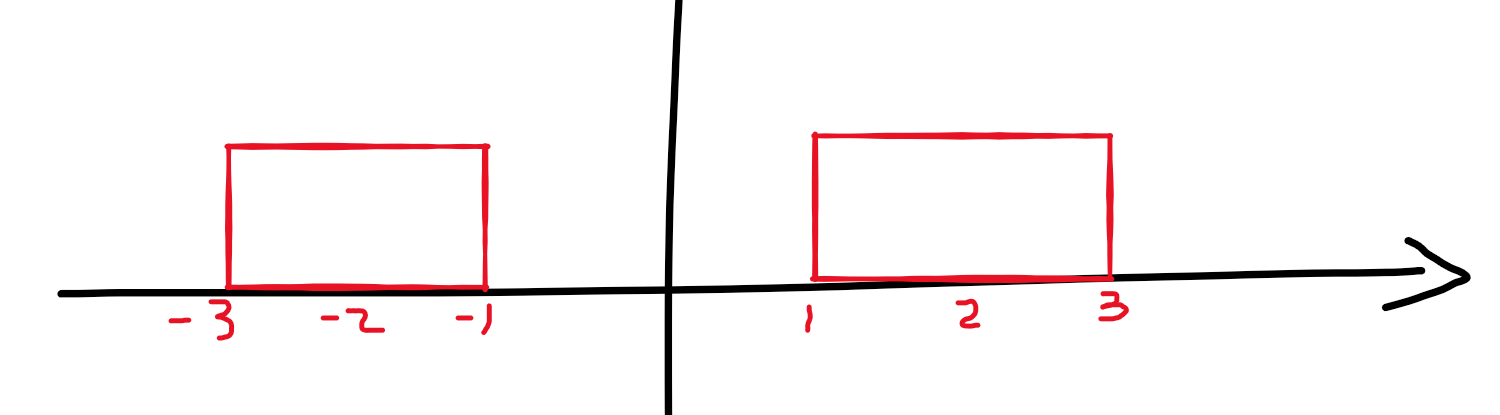该图像可以表示成一个门信号一个门信号,即

g

6

(

w

)

−

g

2

(

w

)

g_6(w)-g_2(w)

,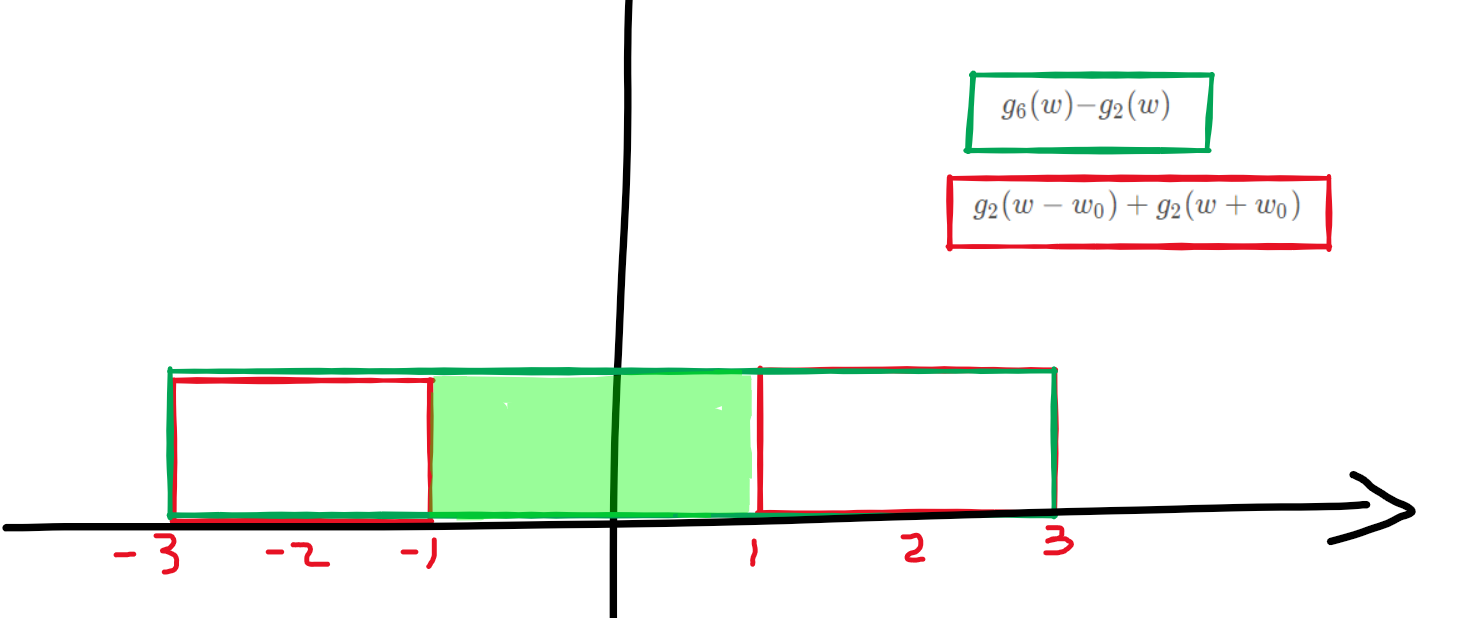此时再做逆变换将会简单一些,大此时化简需要用三角函数的和差化积公式.

三角信号

τ

△

2

τ

(

t

)

=

g

τ

(

t

)

∗

g

τ

(

t

)

τ△_{2τ}(t)= g_τ(t)*g_τ(t)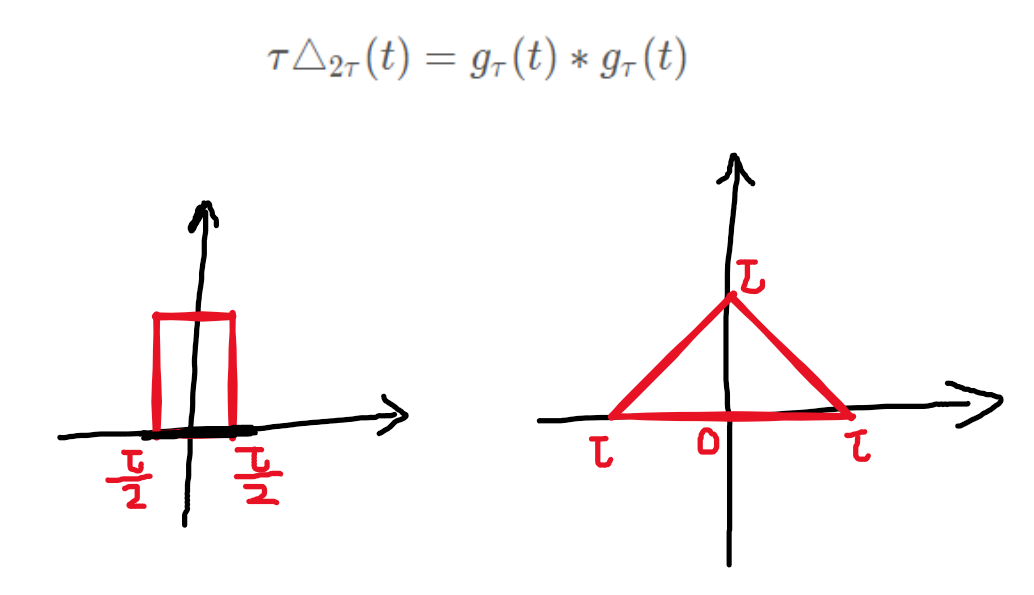1

2

π

g

6

(

w

)

∗

g

6

(

w

)

\frac{1}{2\pi} g_6(w)*g_6(w)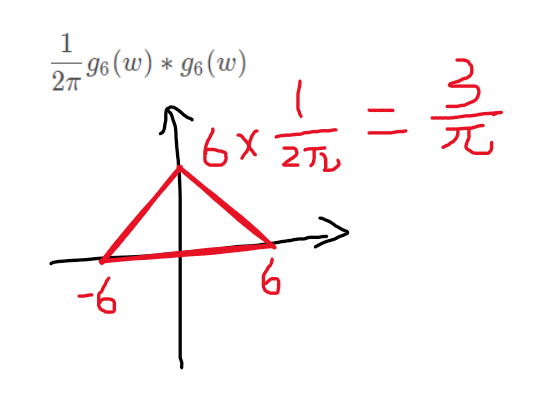希尔伯特变换
遇到与

1

π

t

\frac{1}{\pi t}

卷积说明要利用希尔波特变换

1

π

t

⟷

−

j

s

g

n

(

w

)

\frac{1}{\pi t}\longleftrightarrow-jsgn(w)

与信号

F

(

j

w

)

F(jw)

相乘,[利用了时域相卷频域相乘的性质]
时域-频域-S域-Z域
在时域到频域、(S)(Z)域过程中,若时域中同时出现时移和频移(表现在是否有旋转因子)的情况,要先时移再频移.做逆变换时若同时出现时移和频移,则先频移再时移.在什么域就先做什么域的平移.
时域与频域之间的频谱关系
时域频域连续周期离散非周期连续非周期连续非周期离散周期离散周期离散非周期连续周期
周期信号都做傅里叶级数展开且频谱均离散非周期信号读作傅里叶变换,且频谱均连续时间连续的信号变换到频域为非周期信号时间离散的信号变换到频域为周期信号
计算问题
使用长除法做部分分式展开要注意,分子最高次数大于分母最高次数,当余数最高次数小于分母最高次数时结束计算,此时结果为商+(余数/分母)的形式.

例:

s

3

+

s

2

+

1

(

s

+

1

)

(

s

+

2

)

=

s

3

+

s

2

+

1

s

2

+

3

s

+

2

\frac{s^3+s^2+1}{(s+1)(s+2)}=\frac{s^3+s^2+1}{s^2+3s+2}

分子最高次大于分母最高次,利用长除法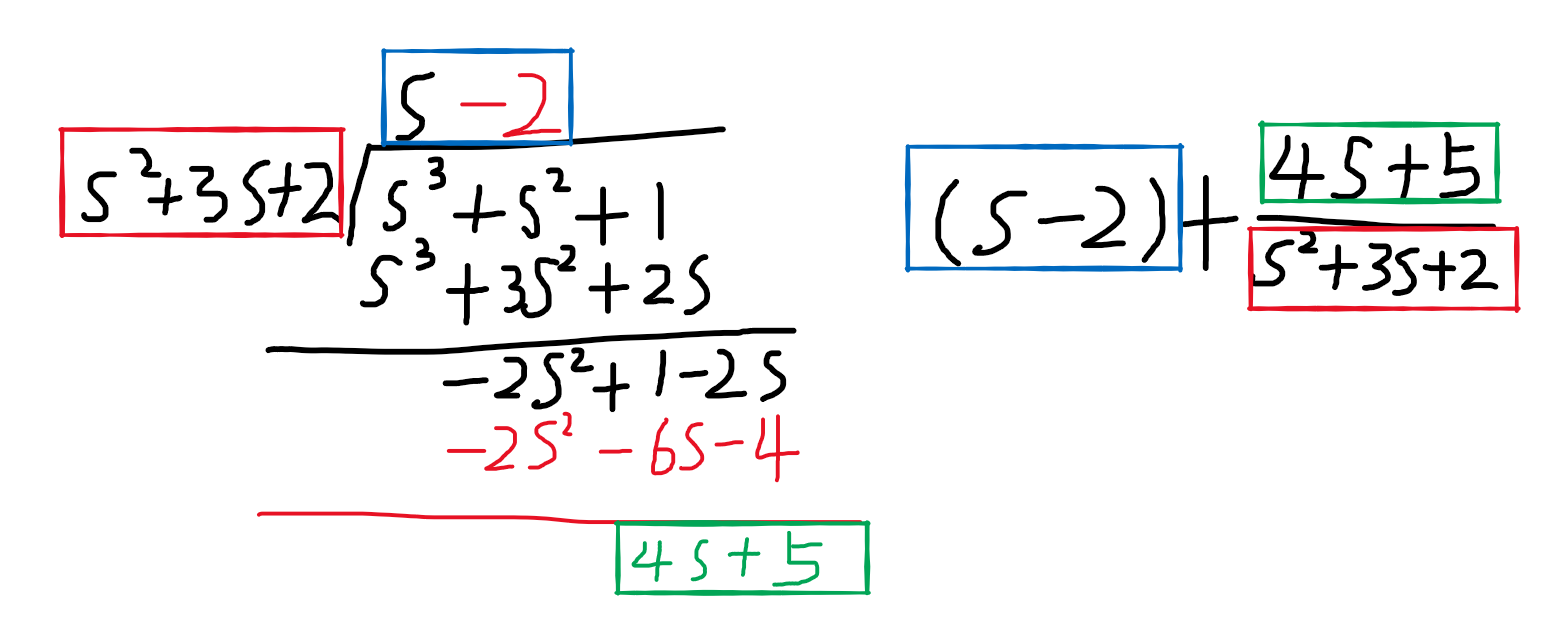(

s

−

2

)

+

4

s

+

5

s

2

+

3

s

+

2

(s-2)+\frac{4s+5}{s^2+3s+2}

做部分分式展开式,当分子分母次数均为1时,可将分子凑成与分母形式一样的式子出现,再做加减拆分,知道分子无变量为止.

例:

1

−

2

s

+

2

s

+

2

+

4

s

+

4

s

−

3

=

1

−

s

+

s

+

2

s

+

2

+

4

(

s

−

3

)

+

4

+

12

s

−

3

1-\frac{2s+2}{s+2}+\frac{4s+4}{s-3}=1-\frac{s+s+2}{s+2}+\frac{4(s-3)+4+12}{s-3}

=

1

−

(

s

s

+

2

+

1

)

+

(

4

+

16

s

−

3

)

=

−

(

s

+

2

−

2

s

+

2

)

+

4

+

16

s

−

3

=1-(\frac{s}{s+2}+1)+(4+\frac{16}{s-3})=-(\frac{s+2-2}{s+2})+4+\frac{16}{s-3}

=

3

+

2

s

+

2

+

16

s

−

3

=3+\frac{2}{s+2}+\frac{16}{s-3}

做逆变换之前查看分子分母是否能凑出可约分项约去.做逆变换时若同时出现时移和频移,则先频移再时移.做单边拉氏变换默认乘以U(t).门信号均用阶跃信号之差来表示.对于

t

U

(

t

−

n

)

tU(t-n)

均化为

(

t

−

n

)

U

(

t

−

n

)

−

n

U

(

t

−

n

)

(t-n)U(t-n)-nU(t-n)

的形式在做变换,其中n为常数.对于

s

i

n

t

U

(

t

−

π

)

sint U(t-\pi)

均化为

s

i

n

(

t

−

π

)

U

(

t

−

π

)

sin(t-\pi)U(t-\pi)

的形式再做变换.
频率响应的滤波特性
输入为

f

(

t

)

=

e

j

w

0

t

f(t)=e^{jw_0t}

,则系统的输出为

y

(

t

)

=

e

j

w

0

t

H

(

j

w

0

)

y(t)=e^{jw_0t}H(jw_0)

输入为

f

(

t

)

=

A

c

o

s

(

w

0

t

+

θ

)

f(t)=Acos(w_0t+\theta )

时,输出为

y

(

t

)

=

A

c

o

s

(

w

0

t

+

θ

+

φ

)

H

(

j

w

0

)

y(t)=Acos(w_0t+\theta+\varphi )H(jw_0)

部分分式展开
F(z)和F(s)做部分分式展开的区别
F(s)满足真分式,即分子最高次小于分母最高次.F(z)满足分子最高次小于或等于分母最高次.F(s)满足条件即可直接展开.F(z)必须要先化成

F

(

z

)

z

\frac{F(z)}{z}

的形式才能展开,且展开后要将

F

(

z

)

z

\frac{F(z)}{z}

还原成F(z),即左右同乘z.
展开技巧
求z逆变换若不是

z

z

+

C

\frac{z}{z+C}

的形式,而是

C

z

\frac{C}{z}

或

z

+

A

B

z

\frac{z+A}{Bz}

的形式,优先化成

A

B

z

\frac{A}{Bz}

的形式,再化成

A

B

z

−

1

+

C

\frac{A}{B}z^{-1}+C

的形式,然后再做逆变换.利用

δ

(

n

)

⟷

1

和

δ

(

n

−

m

)

⟷

z

−

m

\delta(n)\longleftrightarrow 1和\delta(n-m)\longleftrightarrow z^{-m}

形如

F

(

s

)

=

已

知

二

次

方

程

(

S

−

C

)

(

二

次

方

程

)

F(s)=\frac{已知二次方程}{(S-C)(二次方程)}

可分解为

F

(

s

)

=

A

S

−

C

+

B

1

S

+

B

2

二

次

方

程

F(s)=\frac{A}{S-C}+\frac{B_1S+B_2}{二次方程}

的形式. 再利用

A

=

(

S

−

C

)

F

(

s

)

∣

s

=

c

A=(S-C)F(s)|_{s=c}

的方法解出待定系数A,则化为

F

(

s

)

=

已

知

A

S

−

C

+

B

1

S

+

B

2

二

次

方

程

=

已

知

二

次

方

程

(

S

−

C

)

(

二

次

方

程

)

=

F

(

s

)

原

式

F(s)=\frac{已知A}{S-C}+\frac{B_1S+B_2}{二次方程}=\frac{已知二次方程}{(S-C)(二次方程)}=F(s)原式

通过比较

S

,

S

2

S,S^2

等系数解出

B

1

,

B

2

B_1,B_2

后再进行化简或分解.

二

次

方

程

s

2

(

s

+

1

)

\frac{二次方程}{s^2(s+1)}

形式一般可化为

A

S

+

1

+

B

21

S

2

+

B

22

S

\frac{A}{S+1}+\frac{B_{21}}{S^2}+\frac{B_{22}}{S}

的形式 例:

1

4

s

(

s

2

+

1

)

=

1

/

4

s

(

s

2

+

1

)

\frac{1}{4s(s^2+1)}=\frac{1/4}{s(s^2+1)}

一般可拆分为

A

s

+

B

s

+

1

s

2

+

1

\frac{A}{s}+\frac{Bs+1}{s^2+1}

系统稳定性判断
通过系统函数H(s)/H(z)判断系统是否稳定. –1.先求极点 –2.s域:若极点均小于0则稳定.z域:若极点绝对值均小于1则稳定.判断二阶离散系统H(z)的稳定性 朱利准则:对于二阶系统

A

(

z

)

=

a

2

z

2

+

a

1

z

+

a

0

A(z)=a_2z^2+a_1z+a_0

系统稳定的条件是

A

(

1

)

>

0

且

A

(

−

1

)

>

0

且

a

2

>

∣

a

0

∣

A(1)>0且A(-1)>0且a_2>|a_0|

判断二阶连续系统H(s)的稳定性 劳斯霍尔维茨准则:对于二阶系统

A

(

s

)

=

a

2

s

2

+

a

1

s

+

a

0

A(s)=a_2s^2+a_1s+a_0

的全部系数皆为正数且不为0时系统稳定.在S平面虚轴上存在一阶极点和其余极点位于S平面左半开平面时系统为临界稳定.
奈奎斯特

T

s

m

=

1

2

f

m

T_{sm}=\frac{1}{2fm}

:奈奎斯特取样间隔(最大取样间隔).

f

s

m

i

n

=

2

f

m

f_{smin}=2f_m

:奈奎斯特取样率.

w

s

≥

2

w

m

w_s\geq2w_m

:奈奎斯特频率(最小抽样频率).

T

≤

π

w

m

T\leq\frac{\pi}{w_m}

:奈奎斯特间隔.

w

=

2

π

f

w=2\pi f

f

=

w

2

π

f=\frac{w}{2\pi}

2

f

=

w

π

2f=\frac{w}{\pi}

T

=

1

f

T=\frac{1}{f}

响应的初始值求解理解
全响应=零输入响应+零状态响应

y

(

t

)

=

y

z

i

(

t

)

+

y

z

s

(

t

)

y(t)=y_{zi}(t)+y_{zs}(t)

零状态响应在输入的为0时值为0,即在求零输入响应时零状态响应为0.当求零输入响应时,因为零状态响应在输入为0时值为0,所有此时全响应由零输入响应产生.

y

(

t

)

=

y

z

i

(

t

)

y(t)=y_{zi}(t)

当题目给出0_前的初始状态y(0_),若是求零输入响应

y

z

i

(

t

)

y_{zi}(t)

,则因为零输入的输入为0的全响应由零输入产生,故

y

(

0

_

)

=

y

z

i

(

t

)

y(0\_)=y_{zi}(t)

.在求解时需要求解0+后的零输入响应

y

z

i

(

t

)

y_{zi}(t)

,此时需要求出0+后的初始条件

y

z

i

(

0

+

)

y_{zi}(0+)

,因为所求零输入响应

y

z

i

(

t

)

y_{zi}(t)

,故输入为0,即使在0+后输入也依然为0,故零状态响应

y

z

i

(

0

+

)

=

0

y_{zi}(0+)=0

(恒等于0),所以0+后的全响应依然由0+后的零输入响应

y

z

i

(

0

+

)

y_{zi}(0+)

产生.

y

(

0

_

)

=

y

z

i

(

0

−

)

+

0

y(0\_)=y_{zi}(0-)+0

y

(

0

+

)

=

y

z

i

(

0

+

)

+

0

y(0+)=y_{zi}(0+)+0

例:设题目给出的微分方程为

y

′

′

(

t

)

+

A

y

′

(

t

)

+

B

y

(

t

)

=

f

(

t

)

,

求

零

输

入

响

应

y''(t)+Ay'(t)+By(t)=f(t),求零输入响应

因为所求为零输入,故f(t)=0,此时变成齐次方程

y

′

′

(

t

)

+

A

y

′

(

t

)

+

B

y

(

t

)

=

0

y''(t)+Ay'(t)+By(t)=0

对方程两端从0_到0+积分则有

∫

0

−

0

+

y

′

′

(

t

)

d

t

+

A

∫

0

−

0

+

y

′

(

t

)

d

t

+

B

∫

0

−

0

+

y

(

t

)

d

t

=

∫

0

−

0

+

0

d

t

\int_{0-}^{0+}y''(t)dt+A\int_{0-}^{0+}y'(t)dt+B\int_{0-}^{0+}y(t)dt=\int_{0-}^{0+}0dt

根据牛顿莱布尼兹公式

y

′

(

0

+

)

−

y

′

(

0

−

)

+

A

[

y

(

0

+

)

−

y

(

0

−

)

]

+

B

∗

0

=

0

y'(0+)-y'(0-)+A[y(0+)-y(0-)]+B*0=0

因为y(t)从0-到0+积分区域为0(无穷小),故面积为0,也可解释为y(t)在0处无跳变. 为使方程左右两端相等,故有

{

y

′

(

0

+

)

−

y

′

(

0

−

)

=

0

y

(

0

+

)

−

y

(

0

−

)

=

0

\begin{cases} y'(0+)-y'(0-)=0\\ y(0+)-y(0-)=0 \end{cases}

{

y

′

(

0

+

)

=

y

′

(

0

−

)

y

(

0

+

)

=

y

(

0

−

)

\begin{cases} y'(0+)=y'(0-)\\ y(0+)=y(0-) \end{cases}

最后可得

{

y

z

i

(

0

+

)

=

y

(

0

+

)

=

y

(

0

−

)

=

y

z

i

(

0

−

)

y

z

i

′

(

0

+

)

=

y

′

(

0

+

)

=

y

′

(

0

−

)

=

y

z

i

′

(

0

−

)

\begin{cases} y_{zi}(0+)=y(0+)=y(0-)=y_{zi}(0-)\\ y'_{zi}(0+)=y'(0+)=y'(0-)=y'_{zi}(0-) \end{cases}

零输入响应&零状态响应求解

全

响

应

=

零

输

入

响

应

+

零

状

态

响

应

全响应=零输入响应+零状态响应

连续系统
零输入响应
列特征方程求特征根根据特征根设通解形式在求零输入响应时全响应的的初始条件可以当做0+之后的零输入响应,即

y

(

0

−

)

=

y

z

i

(

0

−

)

=

y

(

0

+

)

=

y

z

i

(

0

+

)

y(0-)=y_{zi}(0-)=y(0+)=y_{zi}(0+)

因为零输入时y_{zs}(0-)=0(恒等于),且y(0+)=y(0-).代入初始条件解出通解的常数即得到零输入响应.
零状态响应
首先要知道,求零状态响应时在0-前未输入的情况下y_{zs}(0-)=0(恒等于).对原方程两端从0-到0+积分,会出现

y

′

(

0

+

)

−

y

′

(

0

−

)

,

y

(

0

+

)

−

y

(

0

−

)

y'(0+)-y'(0-),y(0+)-y(0-)

等式子,由

y

(

0

+

)

=

y

z

i

(

0

+

)

+

y

z

s

(

0

+

)

y(0+)=y_{zi}(0+)+y_{zs}(0+)

y

(

0

−

)

=

y

z

i

(

0

−

)

+

y

z

s

(

0

−

)

y(0-)=y_{zi}(0-)+y_{zs}(0-)

可知

y

(

0

+

)

−

y

(

0

−

)

=

y

z

i

(

0

+

)

+

y

z

s

(

0

+

)

−

y

z

i

(

0

−

)

−

y

z

s

(

0

−

)

y(0+)-y(0-)=y_{zi}(0+)+y_{zs}(0+)-y_{zi}(0-)-y_{zs}(0-)

因为在零输入响应时零状态为0,且因为输入为0,故

y

z

i

(

0

+

)

=

y

z

i

(

0

−

)

y_{zi}(0+)=y_{zi}(0-)

上述式子可写为

y

(

0

+

)

−

y

(

0

−

)

=

y

z

s

(

0

+

)

−

y

z

s

(

0

−

)

y(0+)-y(0-)=y_{zs}(0+)-y_{zs}(0-)

又因在零状态时0-前未输入,所以

y

z

s

(

0

−

)

=

0

y_{zs}(0-)=0

,故积分所求结果为

y

z

s

(

0

+

)

y_{zs}(0+)

的值. 上述可推广到

y

z

s

′

(

0

+

)

.

.

.

y

z

s

(

n

)

(

0

+

)

y'_{zs}(0+)...y^{(n)}_{zs}(0+)

根据谁激励的形式设特解,并带入原方程解出特解,并根据特征根列响应的齐次解+特解形式的方程

△

△

.将求出的初始条件

y

z

s

(

0

+

)

.

.

.

y

z

s

(

n

)

(

0

+

)

y_{zs}(0+)...y^{(n)}_{zs}(0+)

代入所设方程

△

△

中,并求出待定常数代回方程

△

△

中,即为零状态响应.
离散系统
零输入响应
根据特征方程求特征根,列通解方程.因为零输入故零状态为零,所以直接代入初始条件

y

(

0

)

.

.

.

y

(

n

)

(

0

)

y(0)...y^{(n)}(0)

解出待定常数.
零状态响应
根据输入激励设相应的特解,并带入原方程解出.根据特征根设齐次解+特解形式的方程

△

△

通过迭代得出零状态响应的初始条件

y

(

0

)

.

.

.

y

(

n

)

y(0)...y(n)

,因为

y

(

n

)

=

y

z

i

(

n

)

+

y

z

s

(

n

)

y(n)=y_{zi}(n)+y_{zs}(n)

故在求解中会因为相减而消去

y

z

i

(

n

)

y_{zi}(n)

,所以迭代出来的

y

(

0

)

.

.

.

y

(

n

)

y(0)...y(n)

,即为

y

z

s

(

0

)

.

.

.

y

z

s

(

n

)

y_{zs}(0)...y_{zs}(n)

将所求的初始条件

y

z

s

(

0

)

.

.

.

y

z

s

(

n

)

y_{zs}(0)...y_{zs}(n)

代入方程

△

△

中解出待定常数再回代,即为零状态响应.
微分方程和差分方程求解注意事项
0+之后的初始状态求法不同.通解的形式不同,特解的形式也不同.写出通解的式子要注明

t

≥

0

,

n

≥

0

t\geq0,n\geq0

写零状态响应要乘以U(t),U(n)表明该方程0之前均为0. 写全响应要注明

t

≥

0

,

n

≥

0

t\geq0,n\geq0

.不能乘以U(t),U(n),因为不知道0之前是否有零输入响应.在求解带特解的方程时:例

y

z

s

(

t

)

=

C

z

s

1

e

−

t

+

C

z

s

2

e

−

3

t

+

y

z

s

p

(

t

)

y_{zs}(t)=C_{zs1}e^{-t}+C_{zs2}e^{-3t}+y_{zsp}(t)

求

y

z

s

′

(

t

)

y'_{zs}(t)

时,因为特解

y

z

s

p

(

t

)

y_{zsp}(t)

也是一个变量,故也要对其进行求导.求零状态初始条件

y

(

0

+

)

y(0+)

时.可能会出现

y

(

0

+

)

−

y

(

0

−

)

=

0

⇒

y

(

0

+

)

=

y

(

0

−

)

y(0+)-y(0-)=0\Rightarrow y(0+)=y(0-)

的情况. 注意此时

y

(

0

−

)

y(0-)

不是题目给定的

y

(

0

−

)

y(0-)

,而是零状态响应

y

z

s

(

0

−

)

y_{zs}(0-)

. 而

y

z

s

(

0

−

)

=

0

(

恒

等

于

)

y_{zs}(0-)=0(恒等于)

由

y

(

0

+

)

=

y

(

0

−

)

⇒

y

z

i

(

0

+

)

+

y

z

s

(

0

+

)

=

y

z

i

(

0

−

)

+

y

z

s

(

0

−

)

y(0+)=y(0-)\Rightarrow y_{zi}(0+)+y_{zs}(0+)=y_{zi}(0-)+y_{zs}(0-)

y

z

s

(

0

+

)

=

y

z

i

(

0

−

)

+

y

z

s

(

0

−

)

−

y

z

i

(

0

+

)

y_{zs}(0+)=y_{zi}(0-)+y_{zs}(0-)-y_{zi}(0+)

激励为输入时,

y

z

i

(

0

+

)

=

y

z

i

(

0

−

)

,

y

z

s

(

0

−

)

=

0

y_{zi}(0+)=y_{zi}(0-),y_{zs}(0-)=0

故

y

z

s

(

0

+

)

=

0

y_{zs}(0+)=0

.求特解时注意不仅把特解代入原方程,其输入也要全部代入.当设特解

y

z

s

p

(

k

)

=

p

0

y_{zsp}(k)=p_0

常数时,

y

z

s

p

(

k

−

n

)

y_{zsp}(k-n)

的差分均为

p

0

p_0

常数.
信号流图

H

(

s

)

=

s

+

2

(

s

+

1

)

(

s

+

3

)

=

s

+

2

s

2

+

4

s

+

3

H(s)=\frac{s+2}{(s+1)(s+3)}=\frac{s+2}{s^2+4s+3}

直接形式(卡尔曼形式)表达式:

H

(

s

)

=

s

−

1

+

2

s

−

2

1

+

4

s

−

1

+

3

s

−

2

H(s)=\frac{s^{-1}+2s^{-2}}{1+4s^{-1}+3s^{-2}}

并联形式表达式:

H

(

s

)

=

0.5

s

+

1

+

0.5

s

+

3

=

0.5

s

−

1

1

+

s

−

1

+

0.5

s

−

1

1

+

3

s

−

1

H(s)=\frac{0.5}{s+1}+\frac{0.5}{s+3}=\frac{0.5s^{-1}}{1+s^{-1}}+\frac{0.5s^{-1}}{1+3s^{-1}}

串联(级联)形式表达式:

H

(

s

)

=

1

s

+

1

⋅

s

+

2

s

+

3

=

s

−

1

1

+

s

−

1

⋅

1

+

2

s

−

1

1

+

3

s

−

1

H(s)=\frac{1}{s+1}·\frac{s+2}{s+3}=\frac{s^{-1}}{1+s^{-1}}·\frac{1+2s^{-1}}{1+3s^{-1}}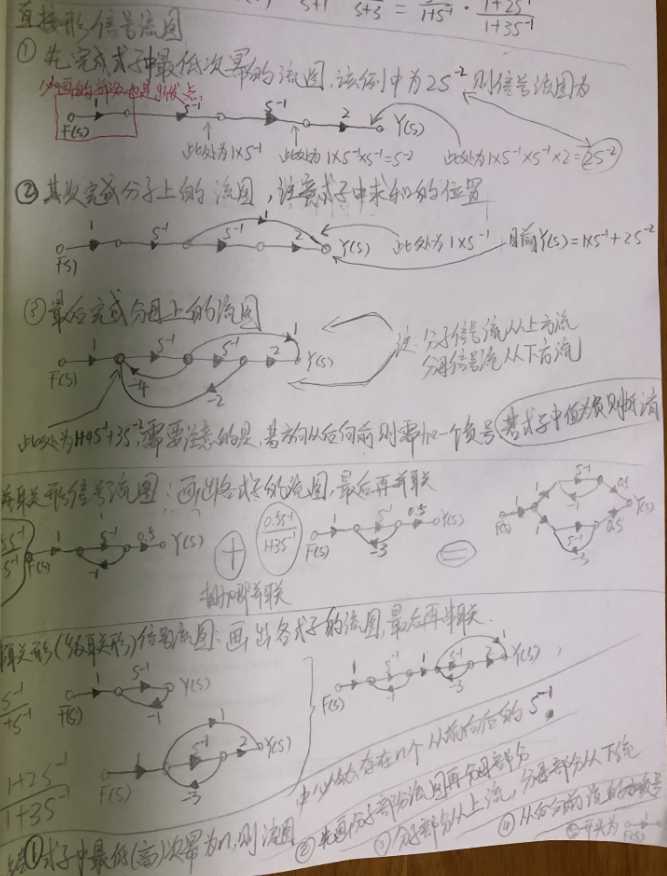在画信号流图时: 1.分解H(s)表达式成需要的形式,注意一般分子次数不大于分母次数. 2.依据分解好的式子画流图,先画流图开头部分,即源点. 3.其次按式中最低次幂,一次增加

s

−

1

s^{-1}

的线段. 4.接着完成分子的流图,其结果输出到最后一个节点,即陷点. 注意:常数也连接到最后节点. 5.最后完成分母部分的流图. 在绘制过程中从后向前流的需增加负号,若值为负则抵消,表现在式中为两式想减的形式.  直接型的式子不需要拆分,其信号流图直接画.  并联型的式子需要部分分式展开,其信号流图分别画各式子的流图,再头与头连,尾与尾连.  串联型的式子(级联型)需拆分成两分式相乘的形式,其信号流图分别画然后依次首尾相连接即可.
系统框图
对于给定系统框图求系统微分方程/差分方程
连续系统
一般设最后一个积分器后面的结果为中间变量. 则每个积分器的前一级为导数关系. 以第一个求和器的结果为基点,列出第一个方程(1式),并化简成左边均为输出,右边均为输入的形式. 以最后一个求和器的结果为基点列出第二个方程(2式),并化简成左边均为输出,右边均为输入的形式. 取(1式)左边输出的式子,并将中间变量替换成y(t)[1式]. 取(2式)右边输入的式子,并将中间变量替换成f(t)[2式]. 令[1式]等于[2式],即得出连续系统的微分方程.
离散系统
一般设第一个差分器(延时器)之前的输入为中间变量. 则每个差分器后为中间变量的差分. 以第一个求和器的结果为基点,列出第一个方程(1式),并并化简成左边均为输出,右边均为输入的形式. 以最后一个求和器的结果为基点列出第二个方程(2式),并化简成左边均为输出,右边均为输入的形式. 取(1式)左边输出的式子,并将中间变量替换成y(k)[1式]. 取(2式)右边输入的式子,并将中间变量替换成f(k)[2式]. 令[1式]等于[2式],即得出离散系统的微分方程.
求解注意
根据系统框图求解冲激响应时切记

f

(

t

)

=

δ

(

t

)

f(t)=\delta(t)

要参与其中.在写方程时需注意系统框图中求和器是否出现负号.
阶跃响应求解
求解阶跃响应时,若h(t)中包含U(t)项,zai对U(t)项积分后记得计算t的取值范围并转换成U(t-n)的形式乘回去.只要对含U(t)的式子进行积分,在积分结束后需计算t的取值范围,并转换为阶跃函数的形式与积分结果相乘.阶跃响应

g

(

t

)

↔

G

(

s

)

g(t)\leftrightarrow G(s)

不是H(s)的导数. 正确的求法为

G

(

s

)

=

H

(

s

)

/

s

=

H

(

s

)

L

[

u

(

t

)

]

G(s)=H(s)/s=H(s)L[u(t)]

然后再逆变换.一般阶跃响应为

e

−

3

t

U

(

t

)

e^{-3t}U(t)

时,求H(s)不考虑求导在拉氏变换,而应直接变换

g

(

t

)

↔

G

(

s

)

g(t)\leftrightarrow G(s)

,再通过

H

(

s

)

=

G

(

s

)

1

/

s

H(s)=\frac{G(s)}{1/s}

来求.
拉普拉斯初值定理和终值定理
初值定理的使用条件
F(s)为真分式(若为假,则化为整数+真分式,然后对真分式使用初值定理)
终值定理的使用条件
F(s)的极点均在左半平面或s=0处只有一阶极点,若不满足则终值

f

(

∞

)

f(∞)

不存在.
初值定理
先将F(s)化简成分子次数小于分母次数(一般用长除法).将F(s)的分母最高次的s的系数化为1(若式子简单也可以不化).若化简后出现整式+真分式的情况,直接舍弃整式,计算真分式的初值定理.
终值定理
先计算极点,若极点均在右半平面或有一阶以上极点在jw轴,则终值

f

(

∞

)

f(∞)

不存在.若时域为发散函数,即收敛域不包含虚轴时终值不存在.
(简易)一元三次方程拆分/求根方法
例:

x

3

+

7

x

2

+

14

x

+

8

=

0

x^3+7x^2+14x+8=0

式中常数8的因子有[1,2,4,8] 为了让因子之和或差等于二次项的系数.故舍弃8.故根为拆分出的因子的相反数.

x

1

=

−

1

,

x

2

=

−

2

,

x

3

=

−

4

x_1=-1, x_2=-2,x_3=-4

即拆分为

(

x

+

1

)

(

x

+

2

)

(

x

+

4

)

=

0

(x+1)(x+2)(x+4)=0

且三个根两两之间的乘积的总和等于一次项系数,即

(

−

1

∗

2

)

+

(

−

1

∗

−

4

)

+

(

−

2

∗

−

4

)

=

14

(-1*2)+(-1*-4)+(-2*-4)=14

拆解步骤
例1:

x

3

+

9

x

2

+

23

x

+

15

=

0

x^3+9x^2+23x+15=0

15的因子为[1,3,5,15]为满足因子之和或差等于二次项的系数,故舍去15取因子相反数,即

x

1

=

−

1

,

x

2

=

−

3

,

x

3

=

−

5

x_1=-1,x_2=-3,x_3=-5

验算:每两根之积的结果的综合是否等于一次项系数

(

−

1

∗

−

3

)

+

(

−

1

∗

−

5

)

+

(

−

3

∗

−

5

)

=

23

(-1*-3)+(-1*-5)+(-3*-5)=23

(

x

+

1

)

(

x

+

3

)

(

x

+

5

)

=

0

(x+1)(x+3)(x+5)=0

例2:

x

3

+

10

x

2

+

27

x

+

18

=

0

x^3+10x^2+27x+18=0

15的因子为[1,2,3,6,9,18]为满足因子之和或差等于二次项的系数且只需保留3个,故舍去9,18,而1+3+6=10,故舍去2取因子相反数,即

x

1

=

−

1

,

x

2

=

−

3

,

x

3

=

−

6

x_1=-1,x_2=-3,x_3=-6

验算:每两根之积的结果的综合是否等于一次项系数

(

−

1

∗

−

3

)

+

(

−

1

∗

−

6

)

+

(

−

3

∗

−

6

)

=

27

(-1*-3)+(-1*-6)+(-3*-6)=27

(

x

+

1

)

(

x

+

3

)

(

x

+

6

)

=

0

(x+1)(x+3)(x+6)=0

重根情况
例3:

x

3

−

4

x

2

+

5

x

−

2

=

0

x^3-4x^2+5x-2=0

2的因子为[1,2]为满足因子之和或差等于二次项的系数且需满足3个,因不足3个则必有重根,为满足因子之和为4,故取[1,2,1]取因子相反数,即\$x_1=-1,x_2=-2,x_3=-1验算:每两根之积的结果的综合是否等于一次项系数

(

−

1

∗

−

2

)

+

(

−

2

∗

−

1

)

+

(

−

1

∗

−

1

)

=

5

(-1*-2)+(-2*-1)+(-1*-1)=5

(

x

+

1

)

2

(

x

+

2

)

=

0

(x+1)^2(x+2)=0

判断是否存在+1,-1的根
考试中一般三次方程会有一个1或-1的根.因为+1,-1是任何数的因子.判断是否有+1,-1的根,即隔次项系数相加等于另一组隔次项系数相加. 例

x

3

+

9

x

2

+

23

x

+

15

=

0

x^3+9x^2+23x+15=0

三次项系数+一次项系数(1+23)=24

[

1

式

]

[1式]

二次项系数+零次项系数(9+15)=24

[

2

式

]

[2式]

因为

[

1

式

]

=

[

2

式

]

[1式]=[2式]

,故必有一个根为(-1)若[1式]和[2式]互为相反数,即

[

1

式

]

=

−

[

2

式

]

[1式]=-[2式]

,则必有一个根为1.
方法失效的情况
当上述方法不起租用说明可能存在共轭根 例

s

3

+

s

2

−

2

=

0

s^3+s^2-2=0

此时

1

+

2

+

1

≠

1

1+2+1\neq1

,且

(

−

1

)

+

(

−

2

)

+

(

−

1

)

≠

1

(-1)+(-2)+(-1)\neq1

, 但判断是否存在

±

1

\pm1

的方法依然有效. 当判断存在一个

±

1

\pm1

根后可用长除法. 因为有一个根为(1),故除数为(s-1) 结果

(

s

−

1

)

(

s

2

+

2

s

+

2

)

(s-1)(s^2+2s+2)

此时再用配方法,十字相乘公式法等化简.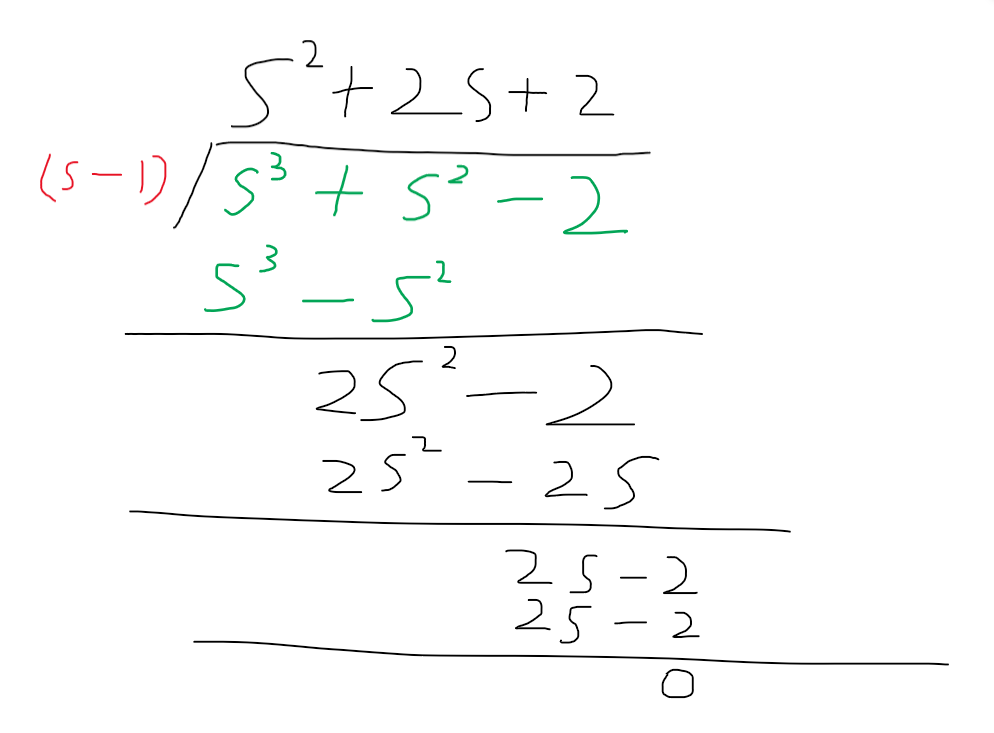展开全文...